# ML Aggarwal Solutions for Class 10 Maths Chapter 1 Goods and Service Tax (GST)

ML Aggarwal Solutions for Class 10 Maths Chapter 1 Goods and Service Tax (GST) are available here. In this chapter, we shall discuss topics based on goods and its service tax. GST is a value-added tax levied on most goods and services sold for domestic consumption. The GST is paid by the consumers, but it is remitted to the government by the businesses selling the goods and services. In effect, GST provides revenue for the government. For better understanding of the concepts, the expert faculty team at BYJU’S have solved the problems based on GST in a step by step manner to help students understand the concepts easily. Students can refer to ML Aggarwal Solutions for Class 10, which is the best reference guide any student can rely on to achieve their goals. Tutors have designed the solutions in a unique and simple method where any student can grasp the topics clearly. The solutions to this chapter are readily available in the pdf format, which can be downloaded by the students easily from the links provided below and can start practising offline to score good marks in their board exams.

ML Aggarwal Solutions for Class 10 Maths Goods and Service Tax (GST) contains one exercise and the ML Aggarwal Class 10 Solutions present in this page provide solutions to the questions discussed in this exercise.

## ML Aggarwal Solutions for Class 10 Maths Chapter 1 – Goods and Service Tax (GST) :-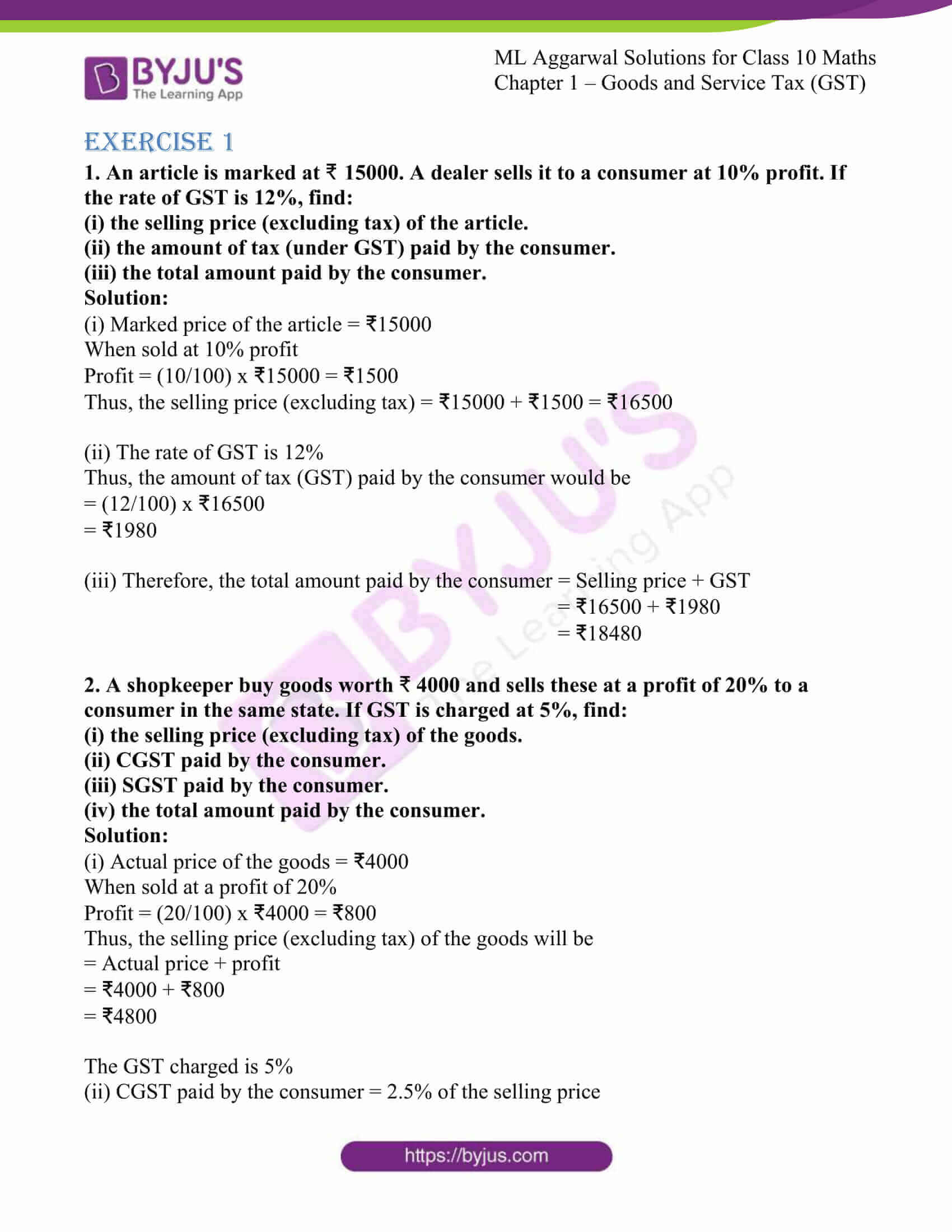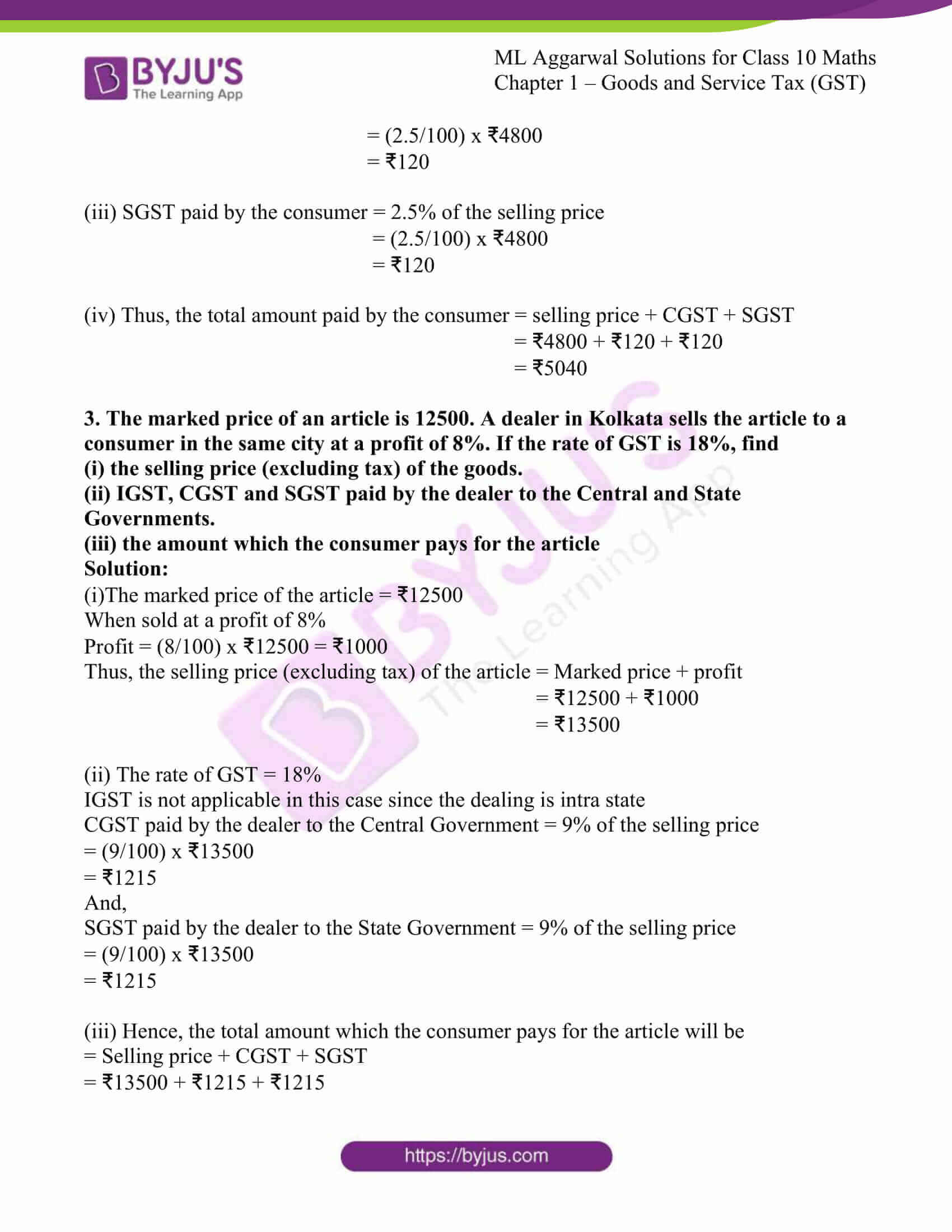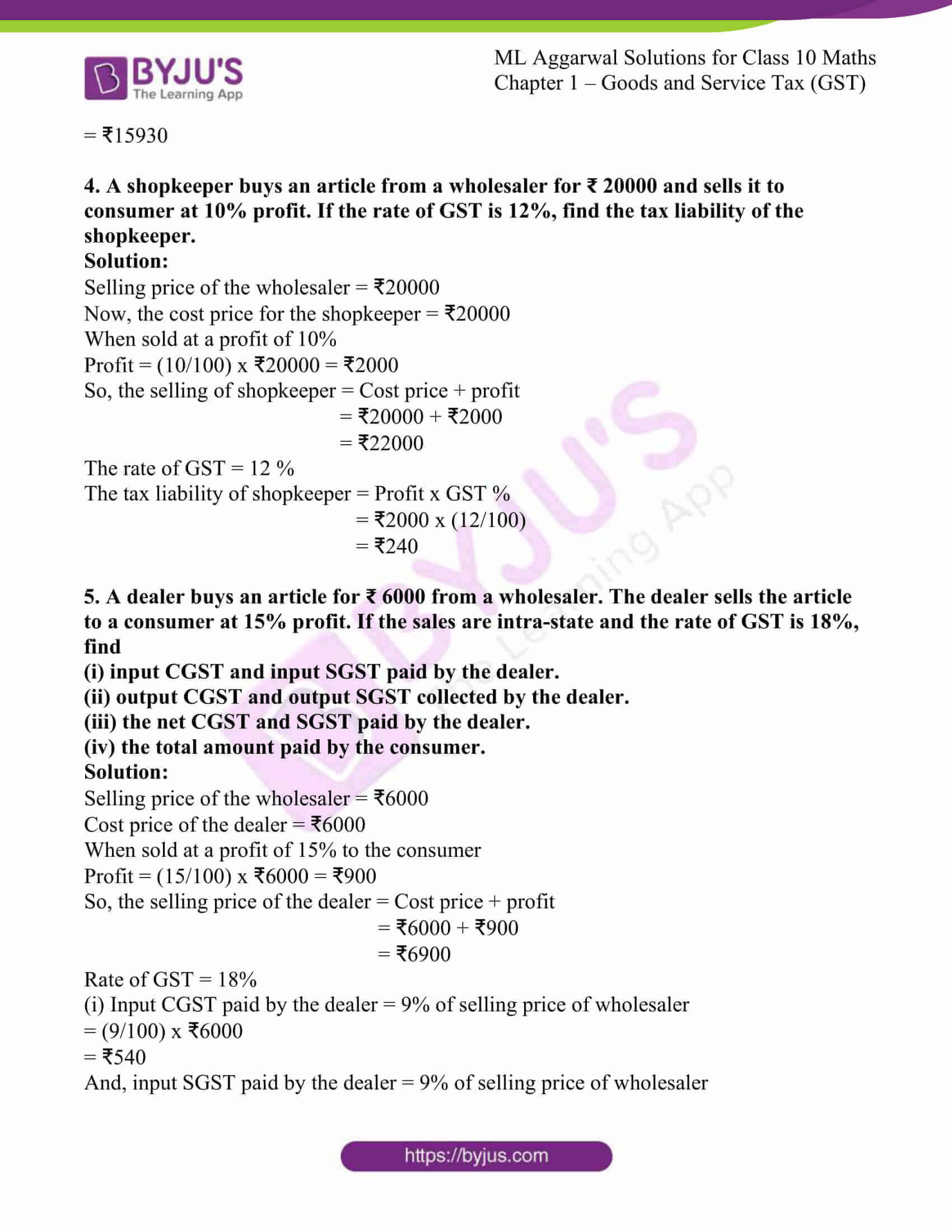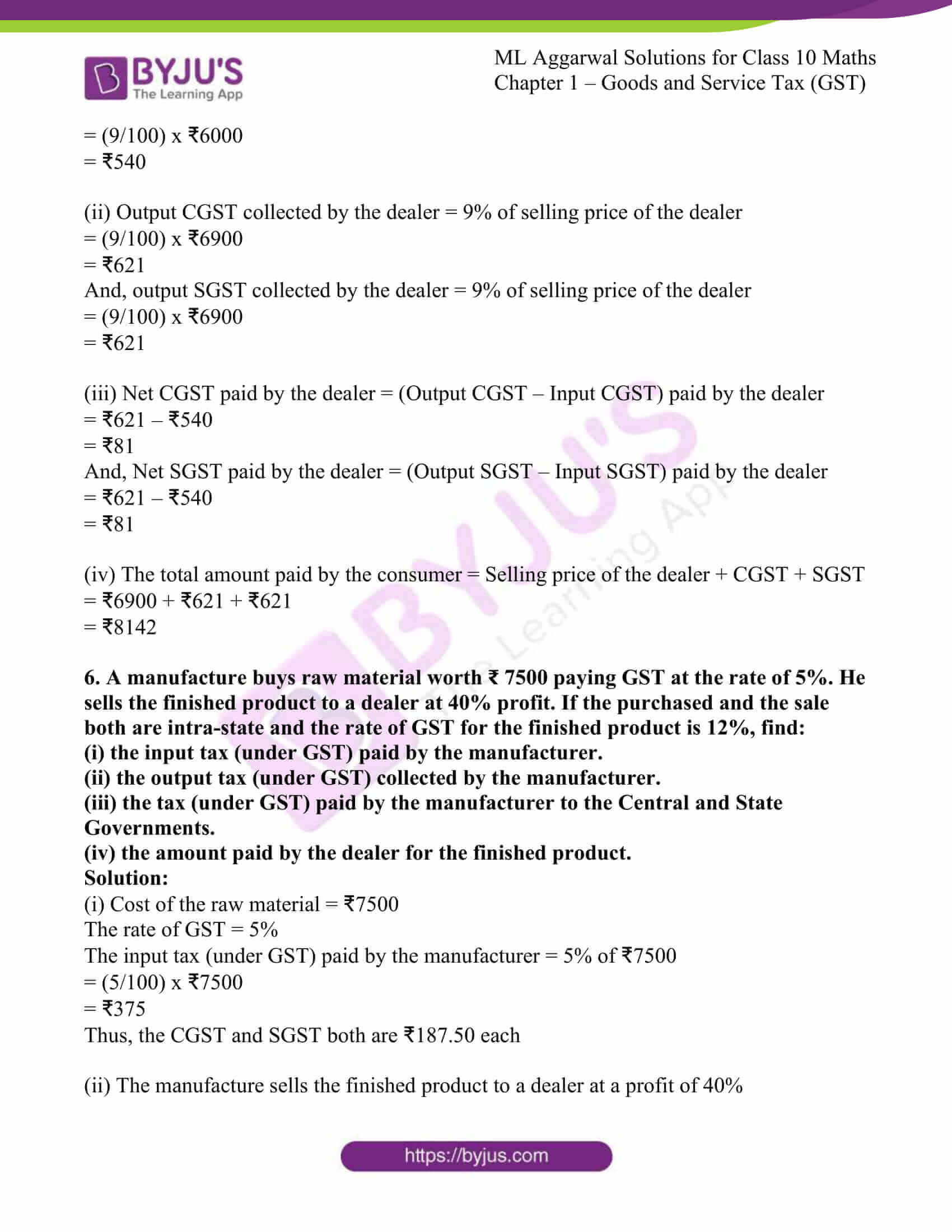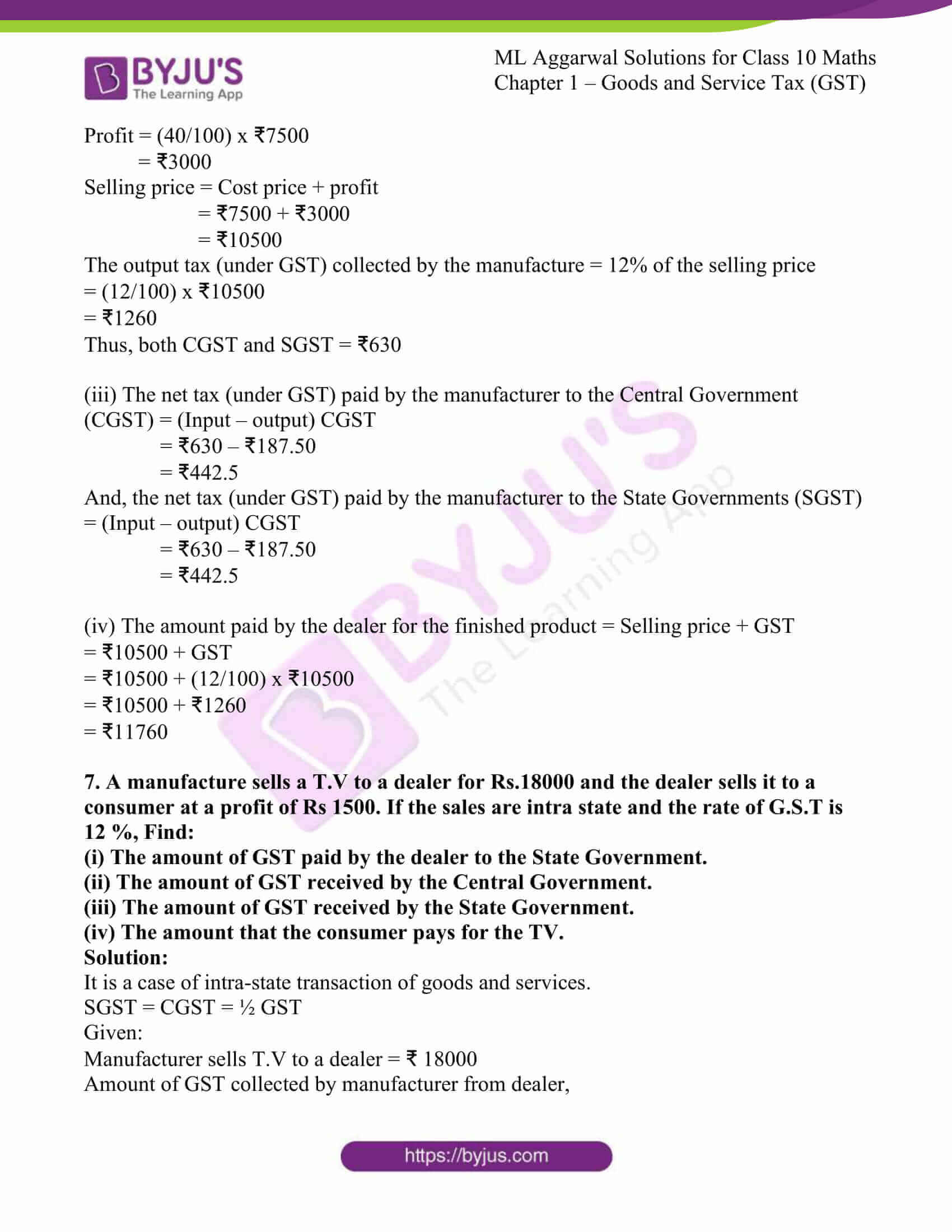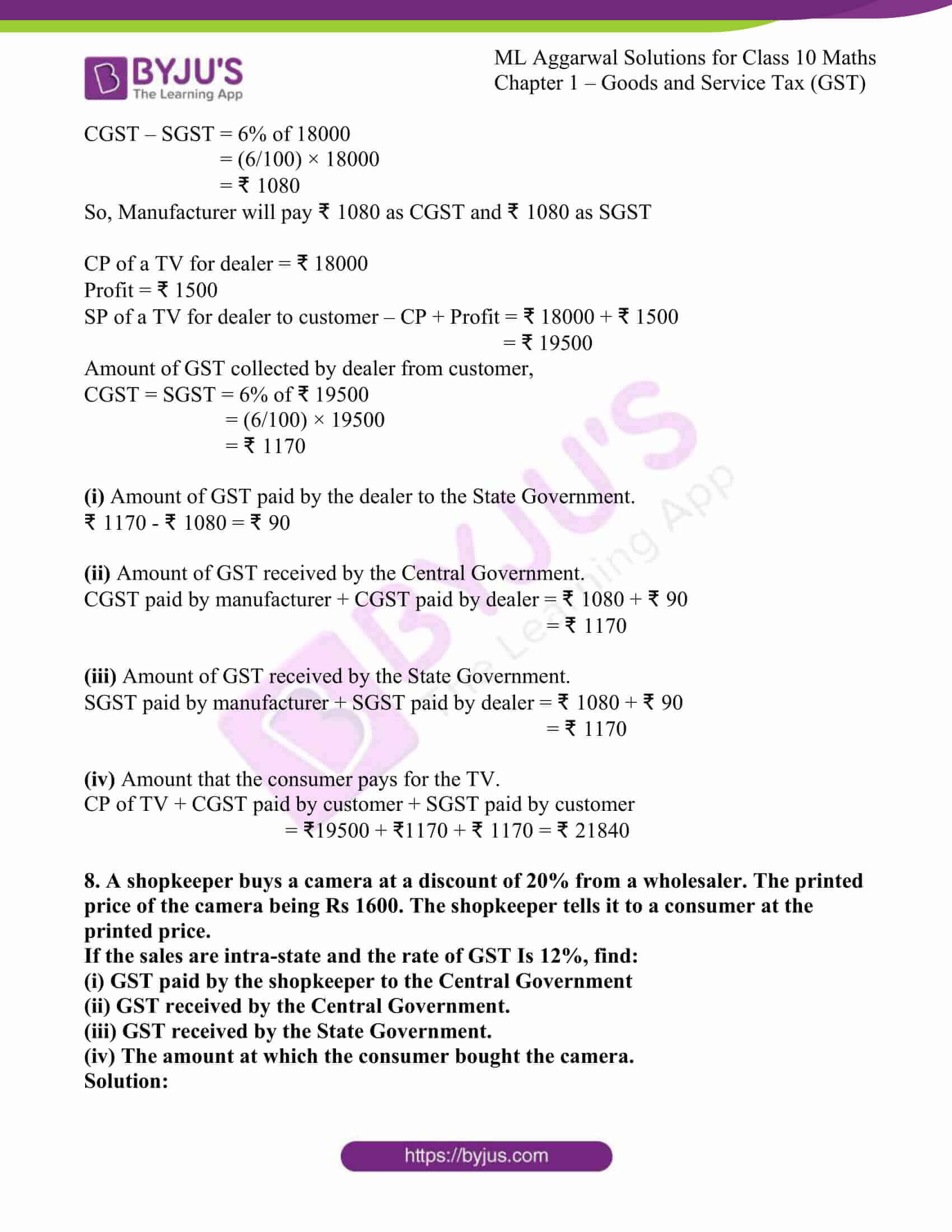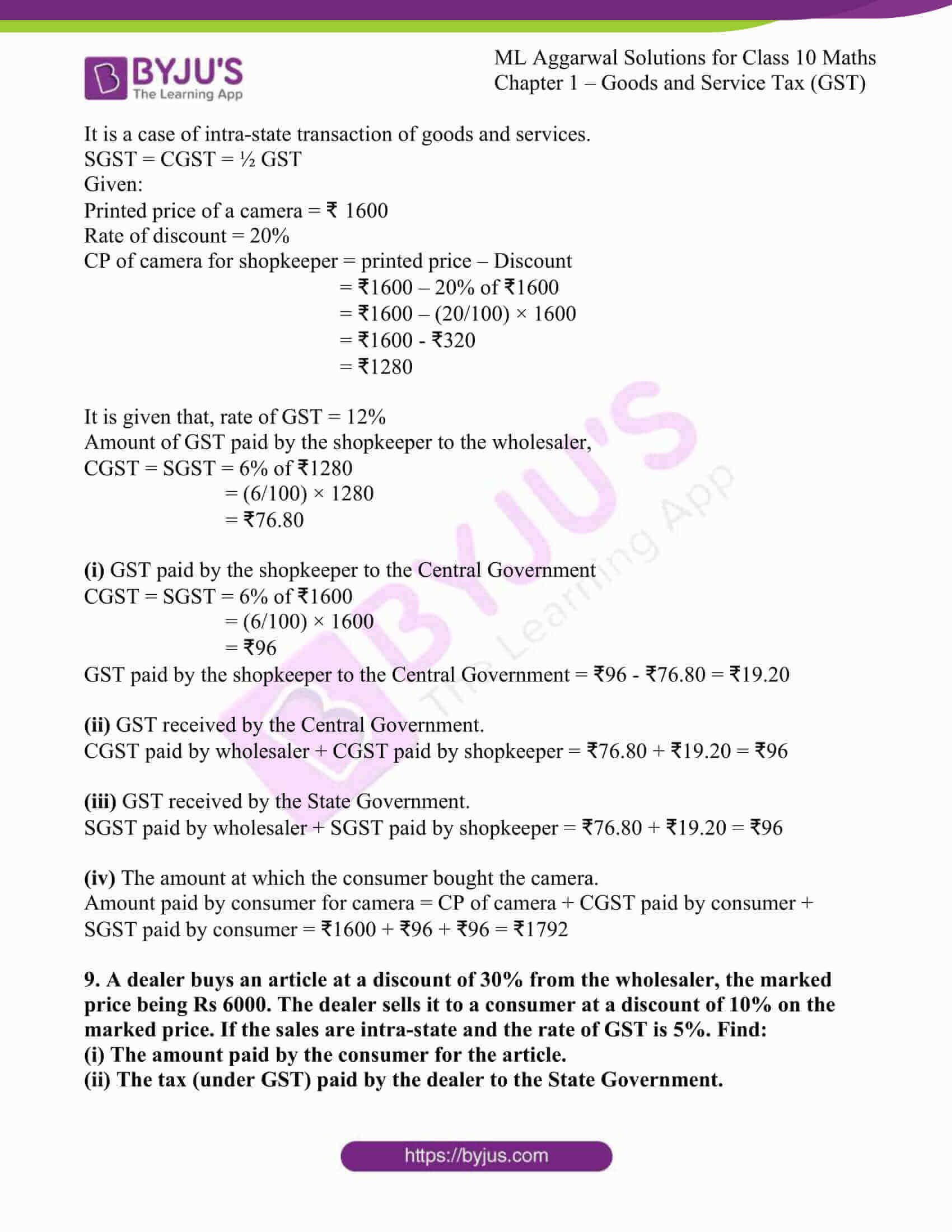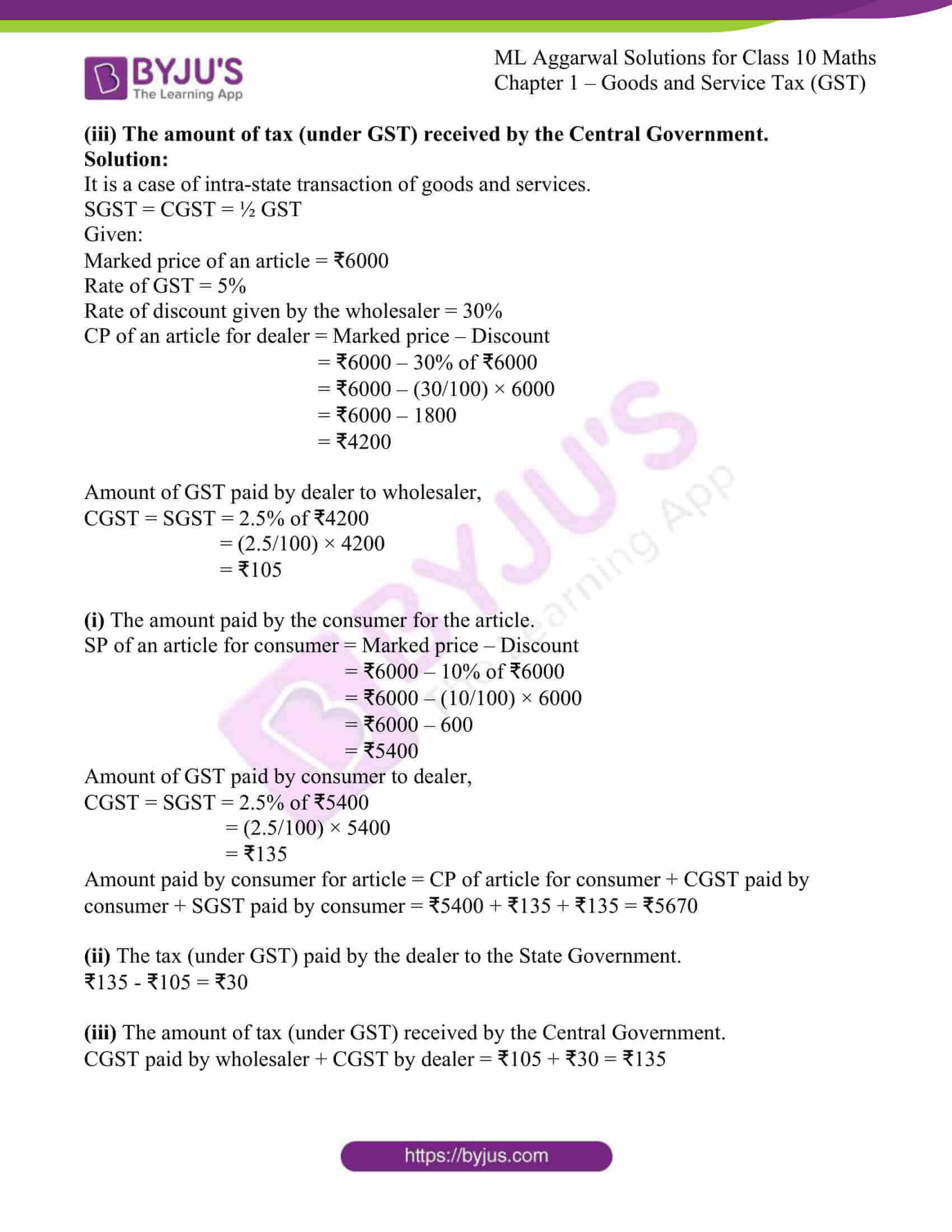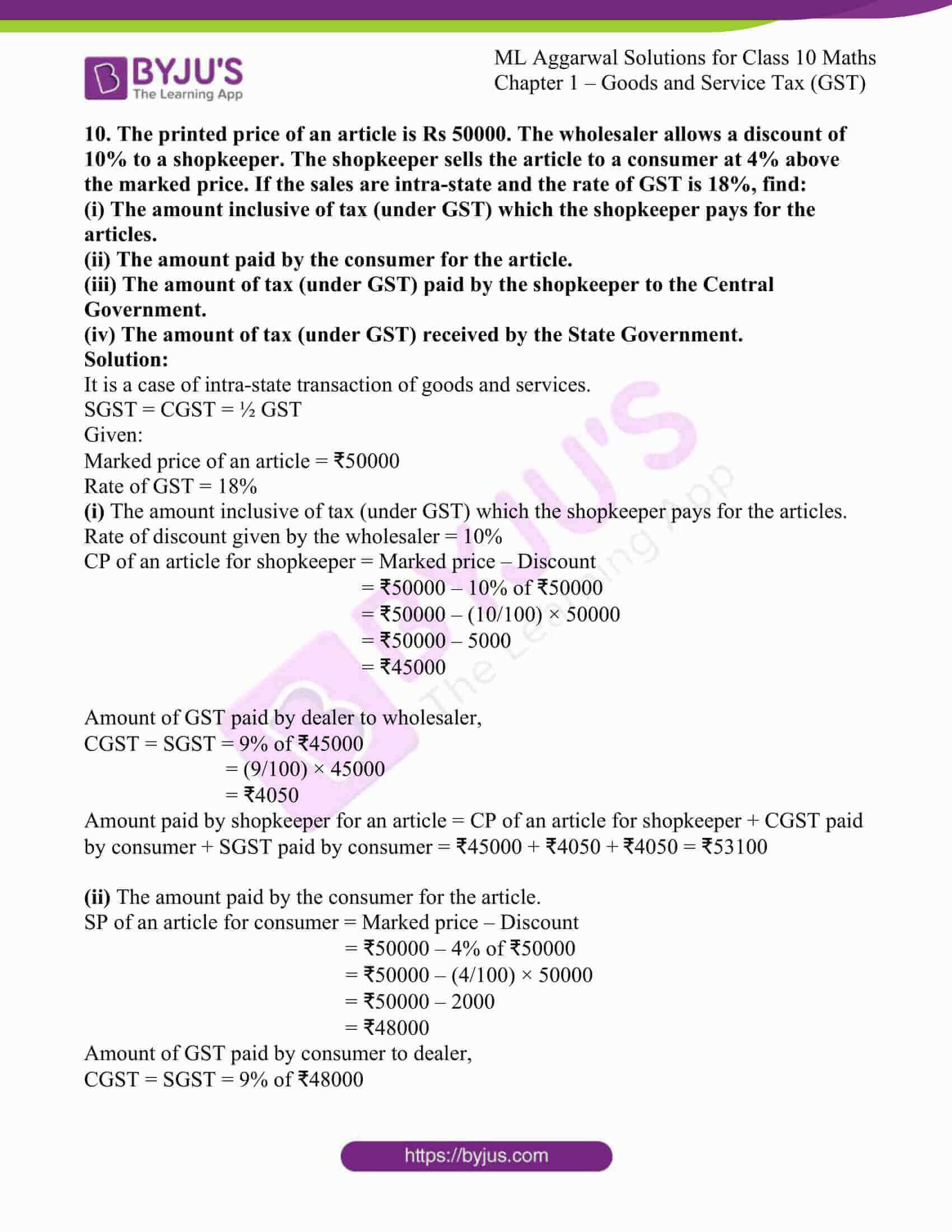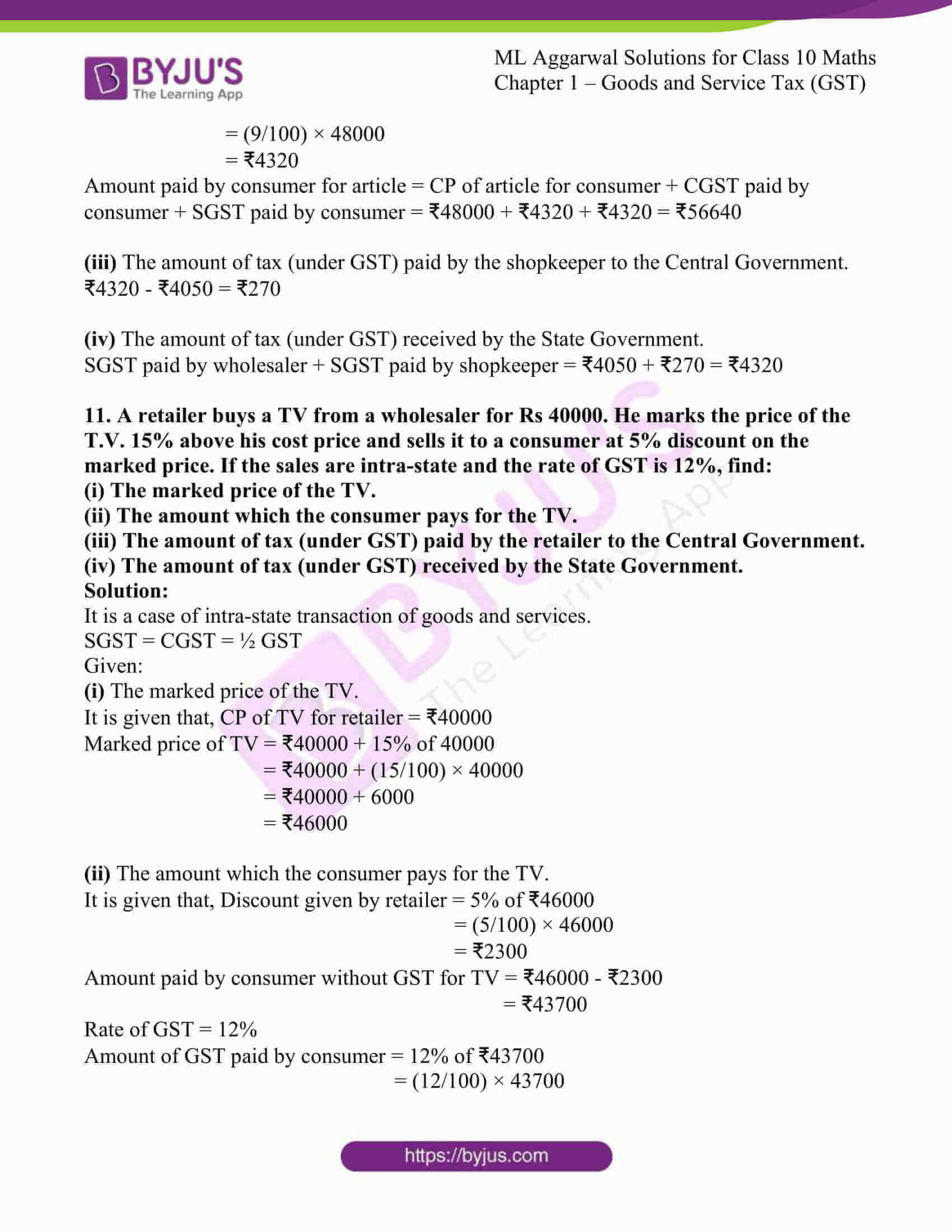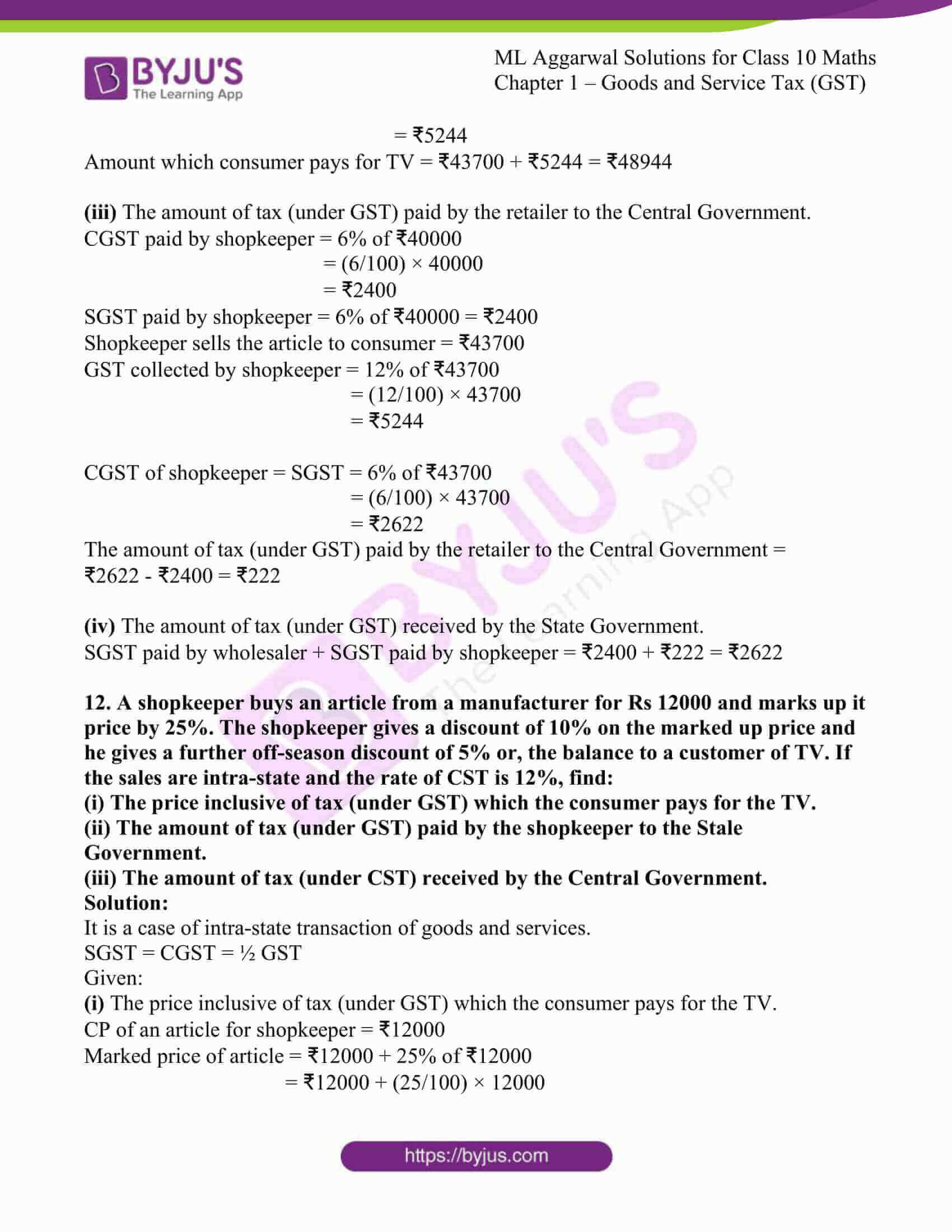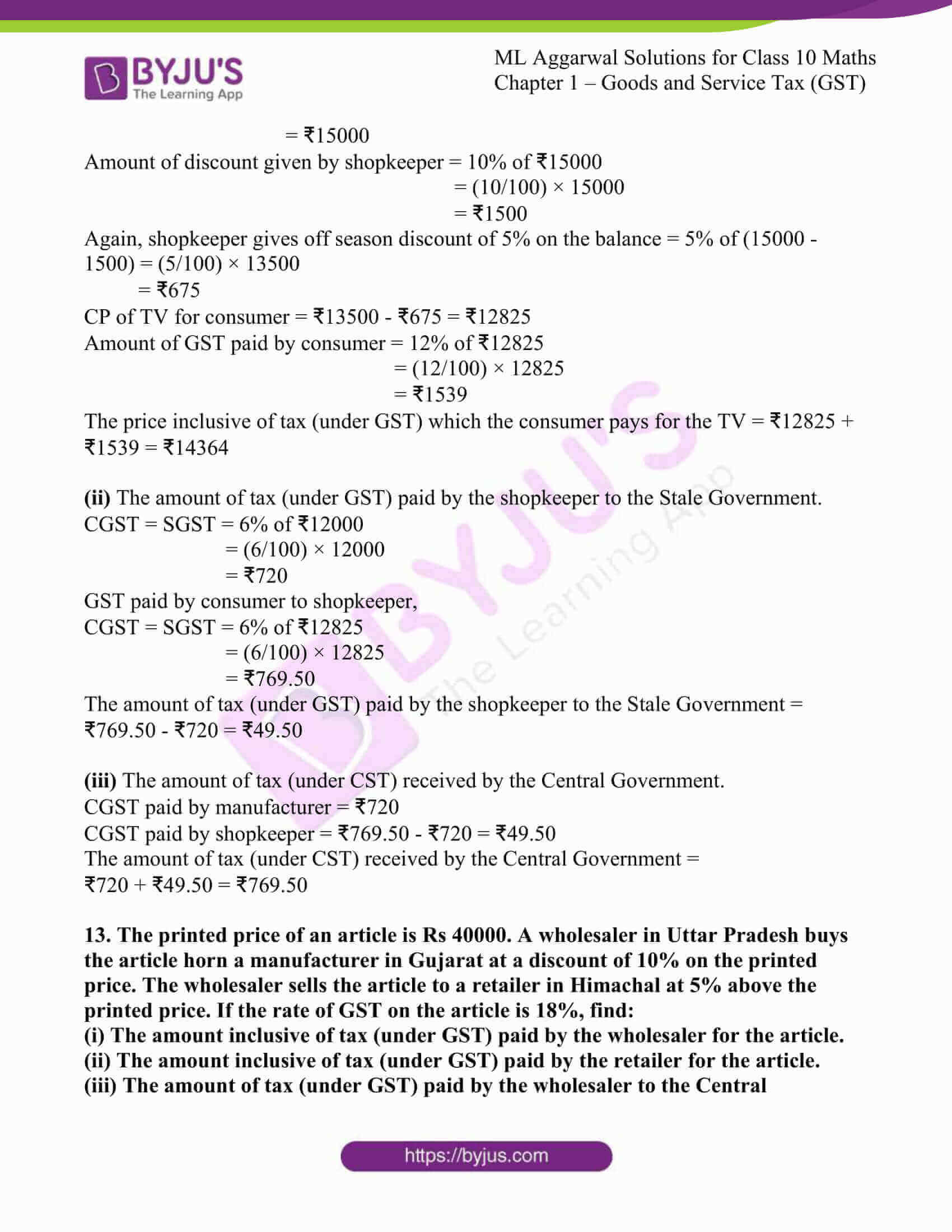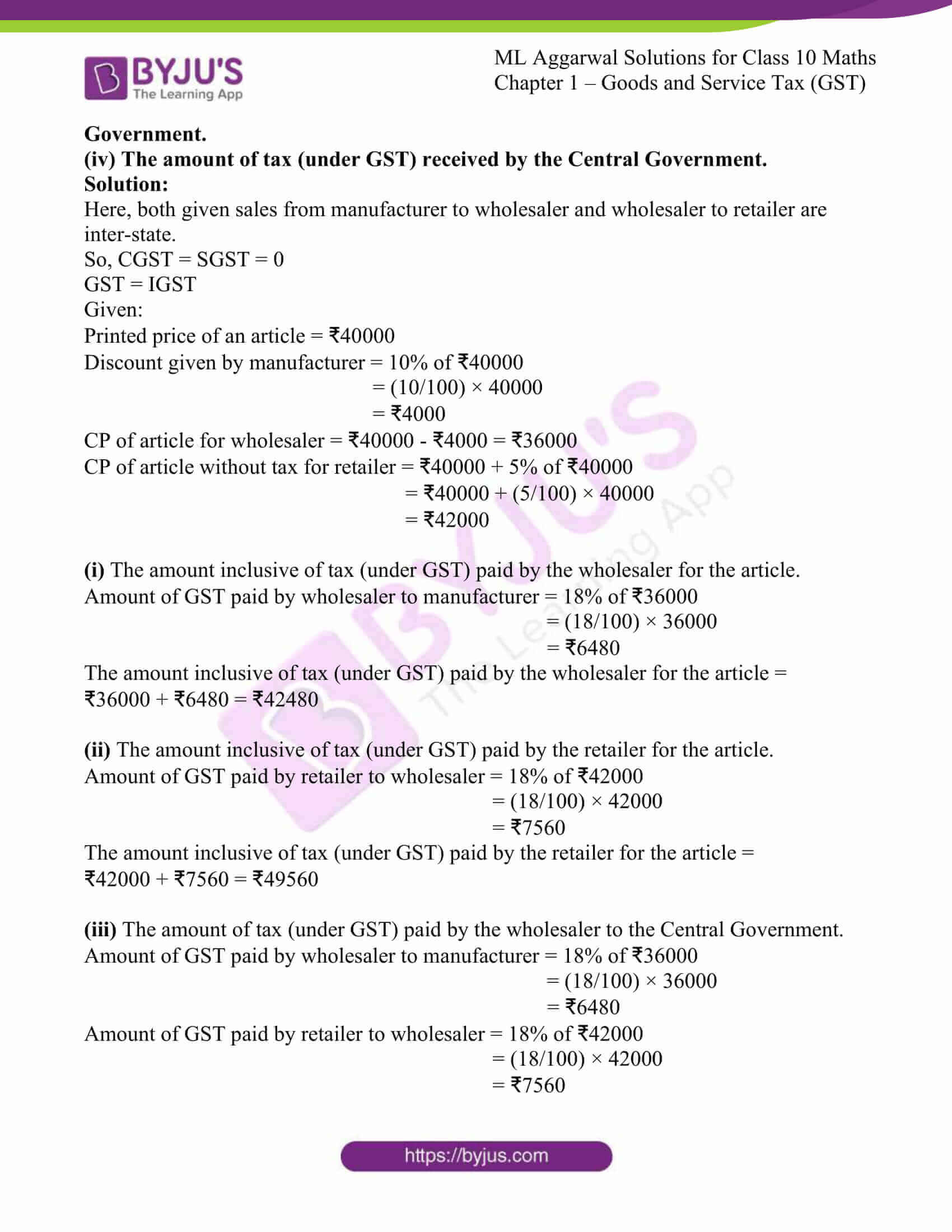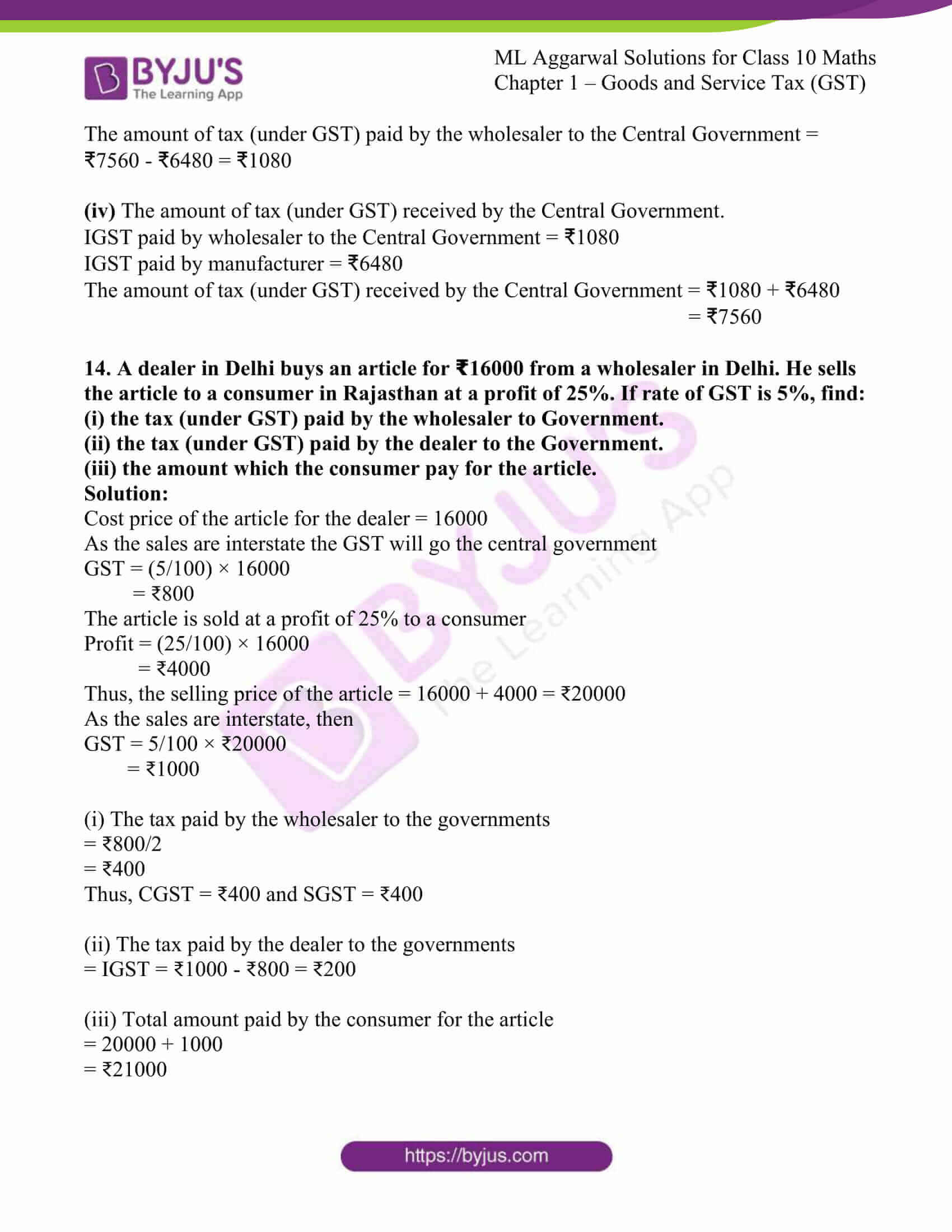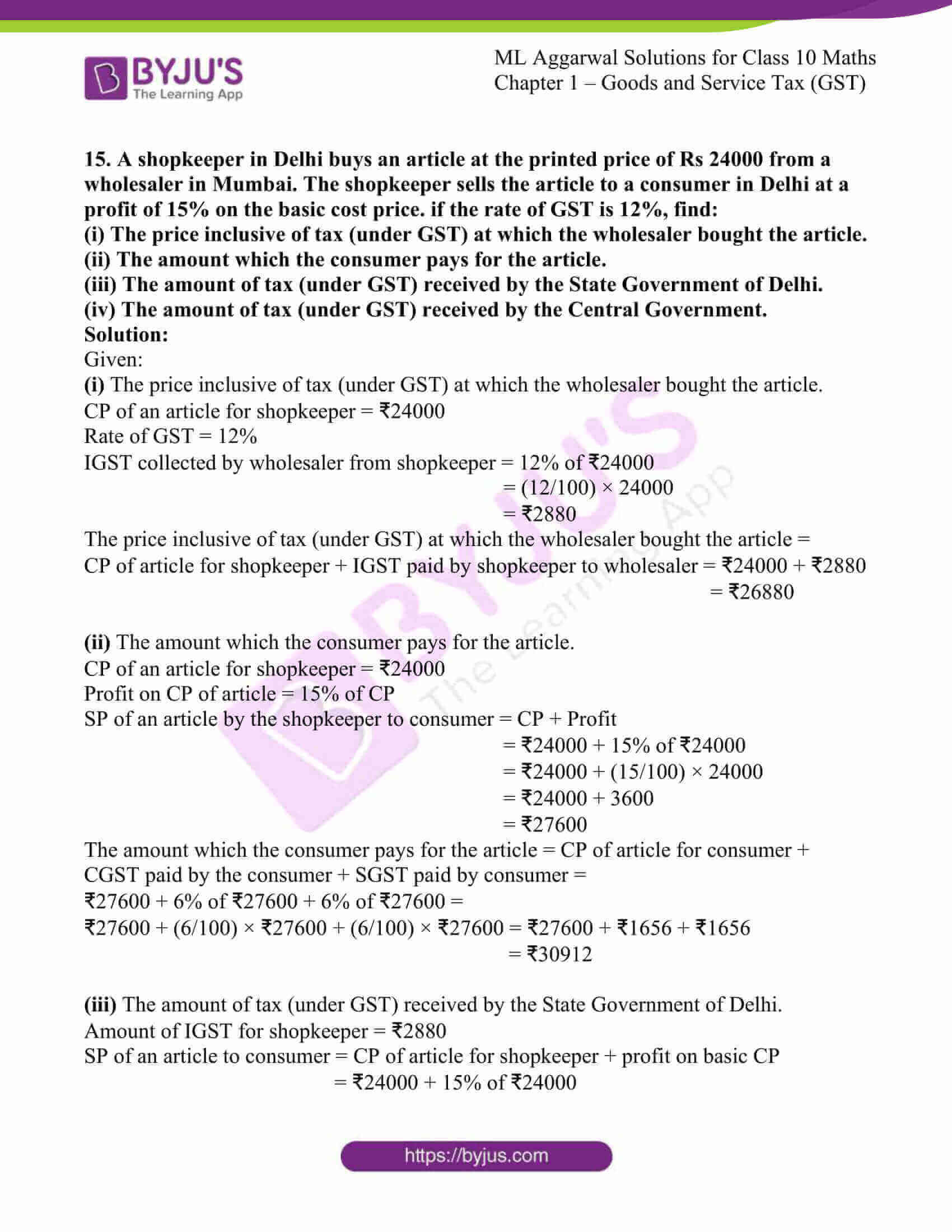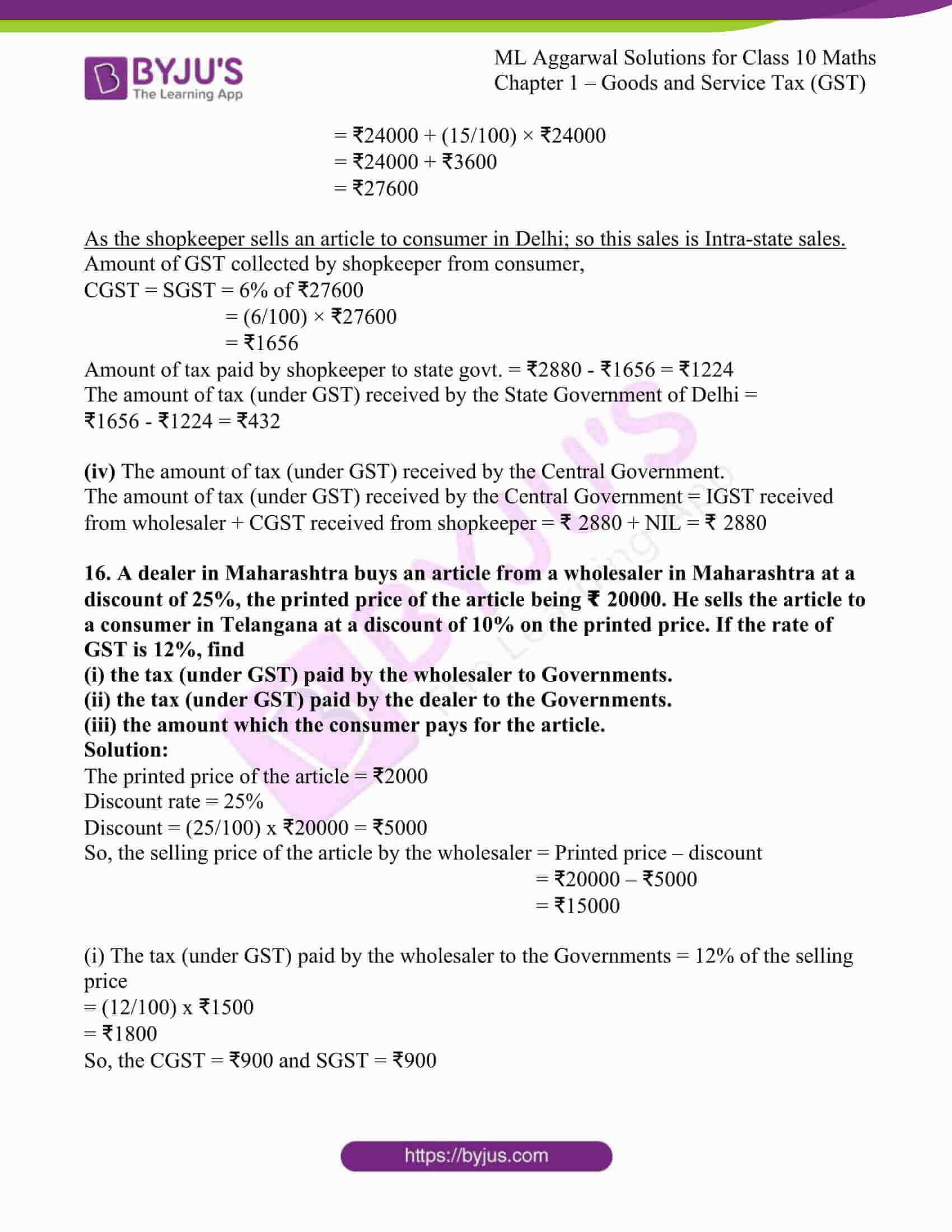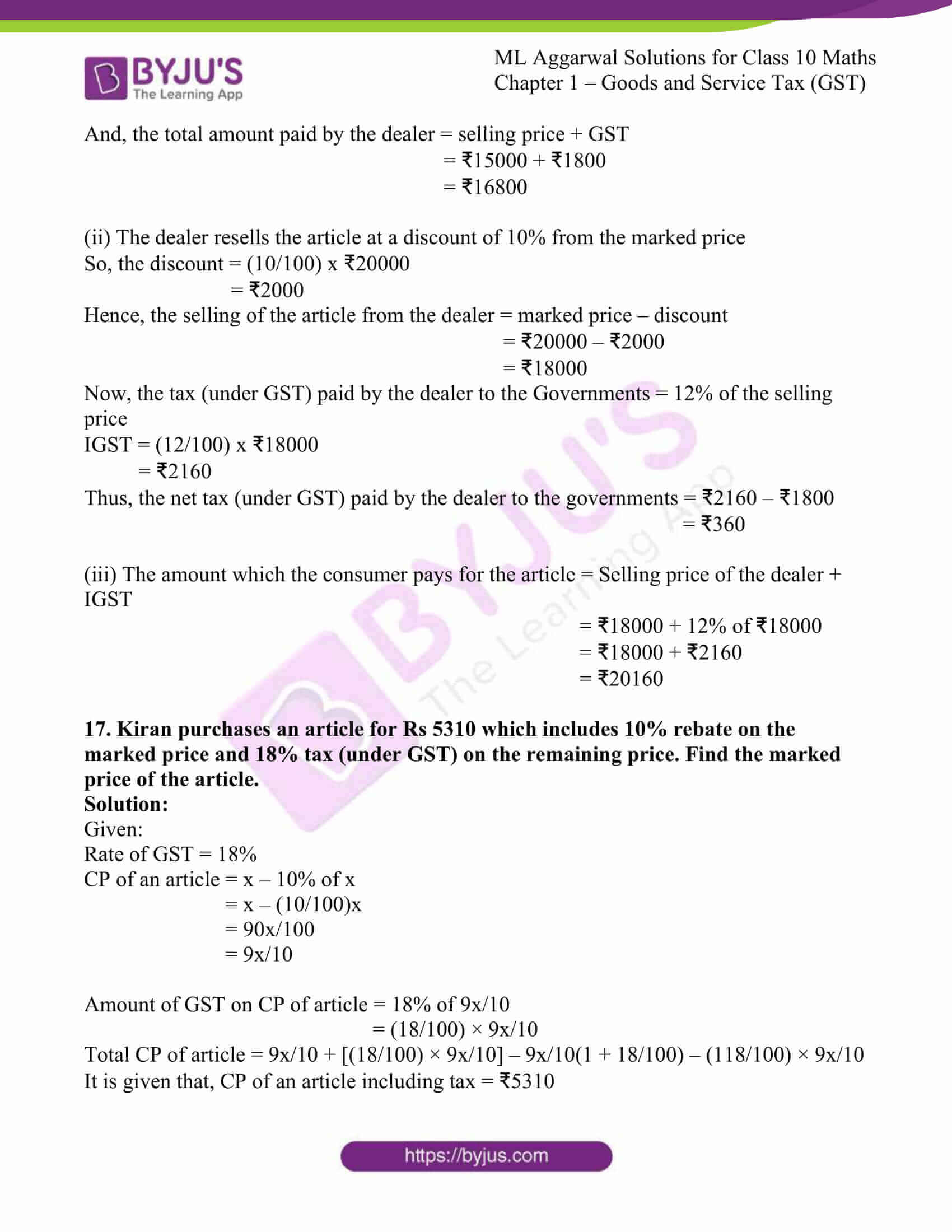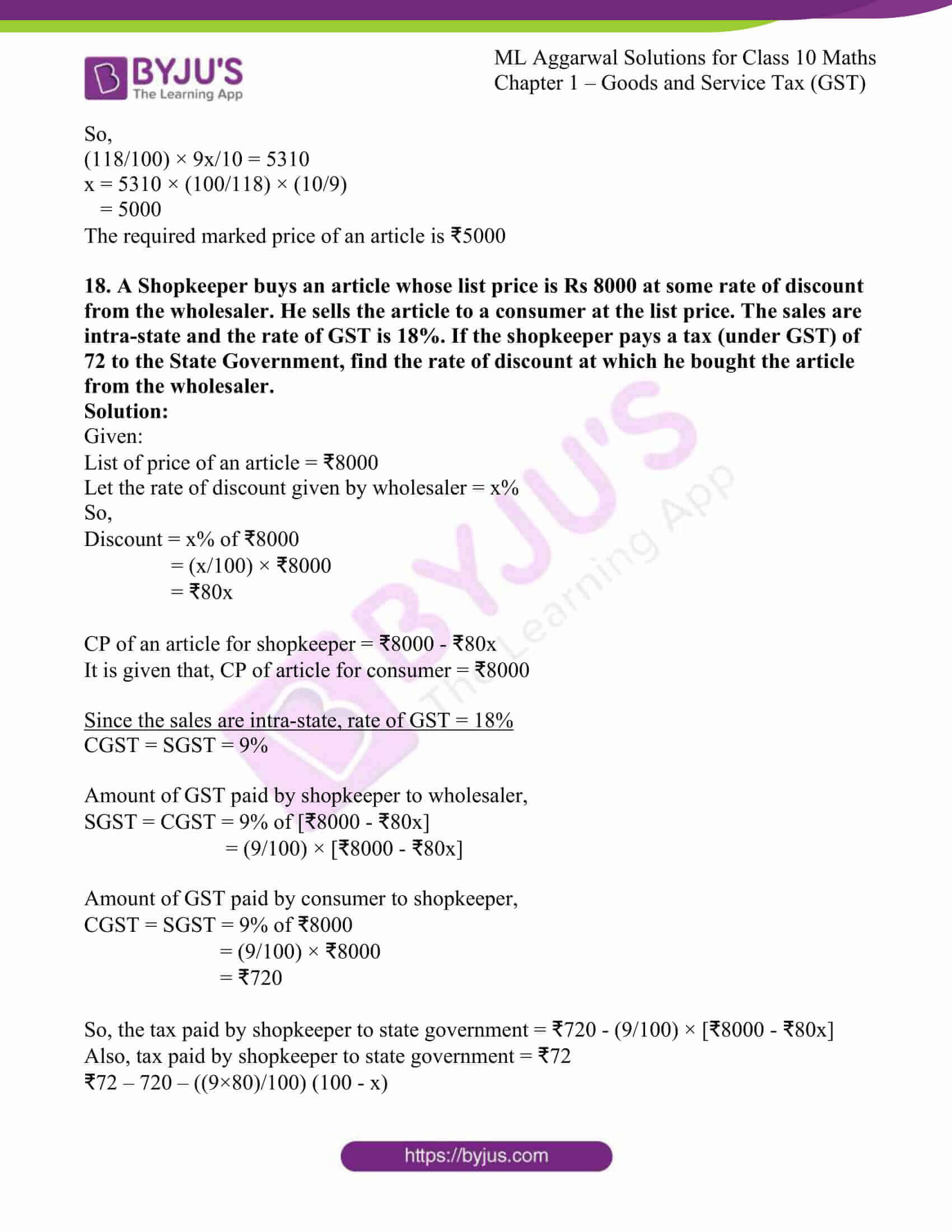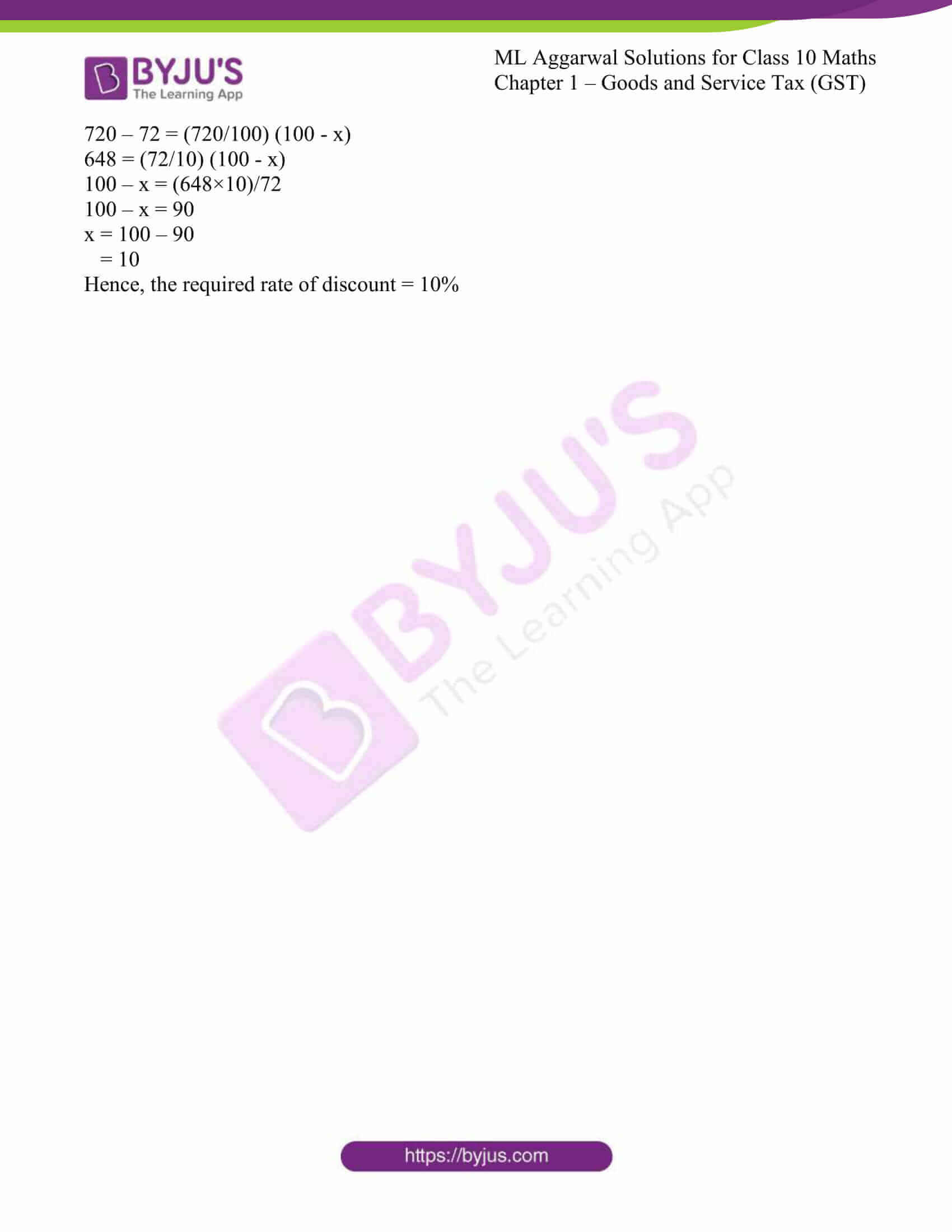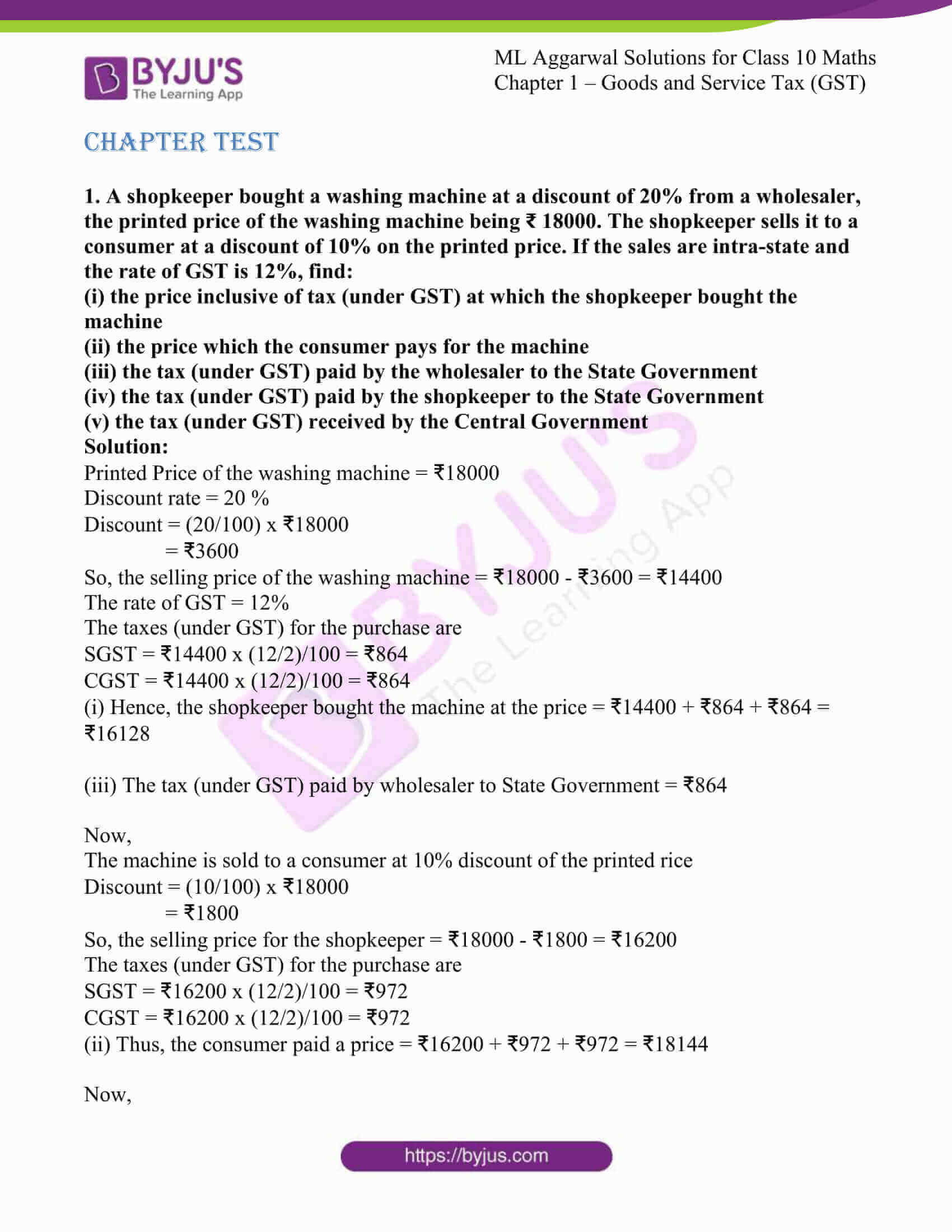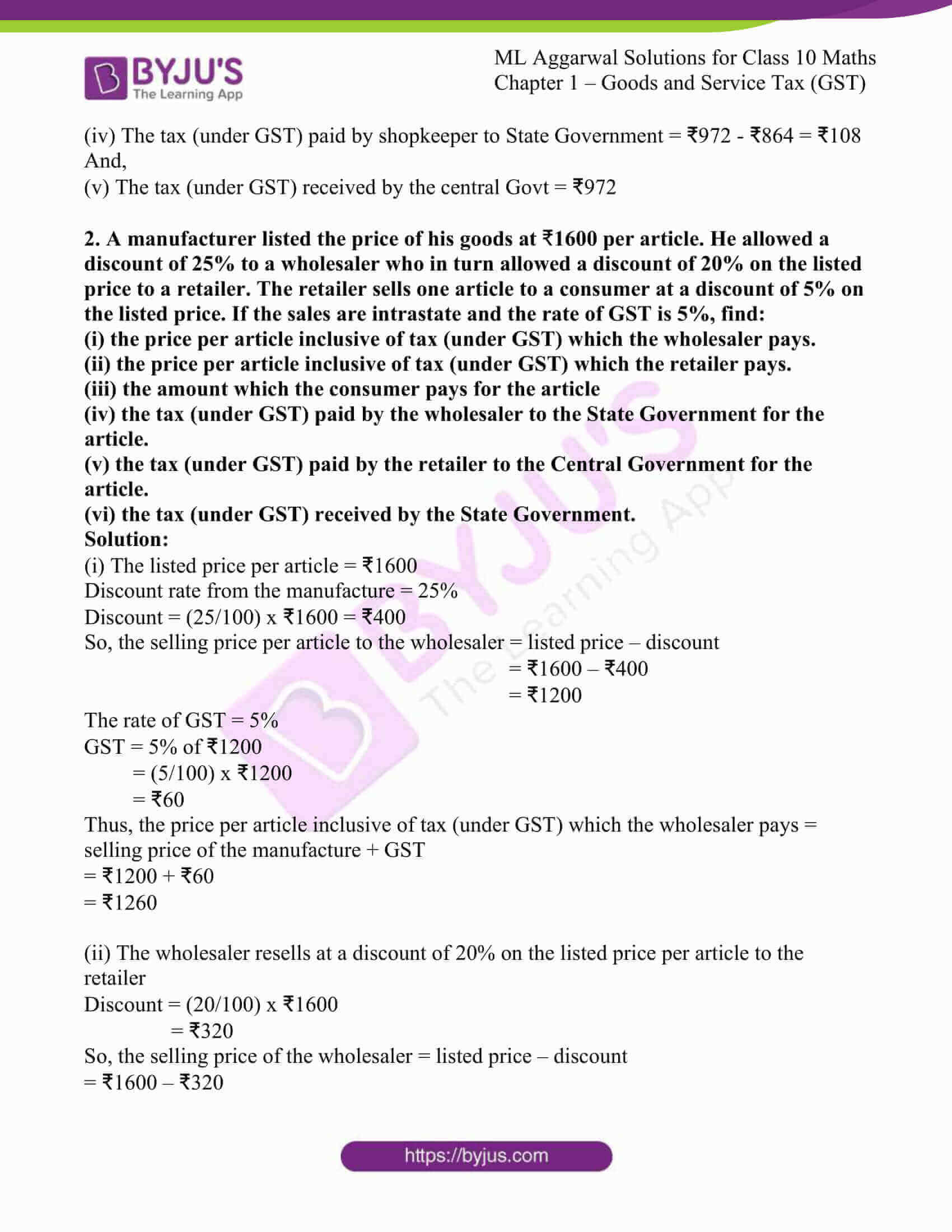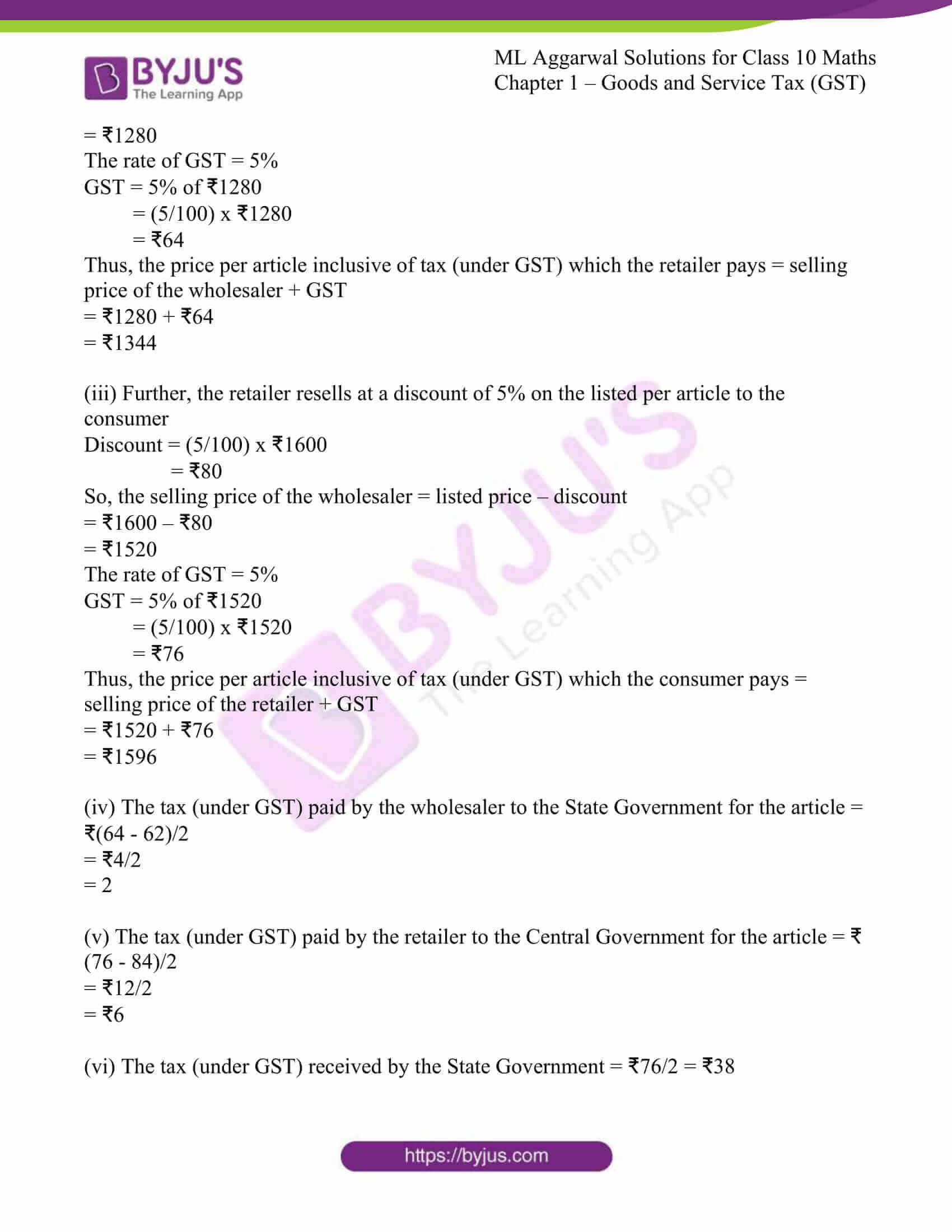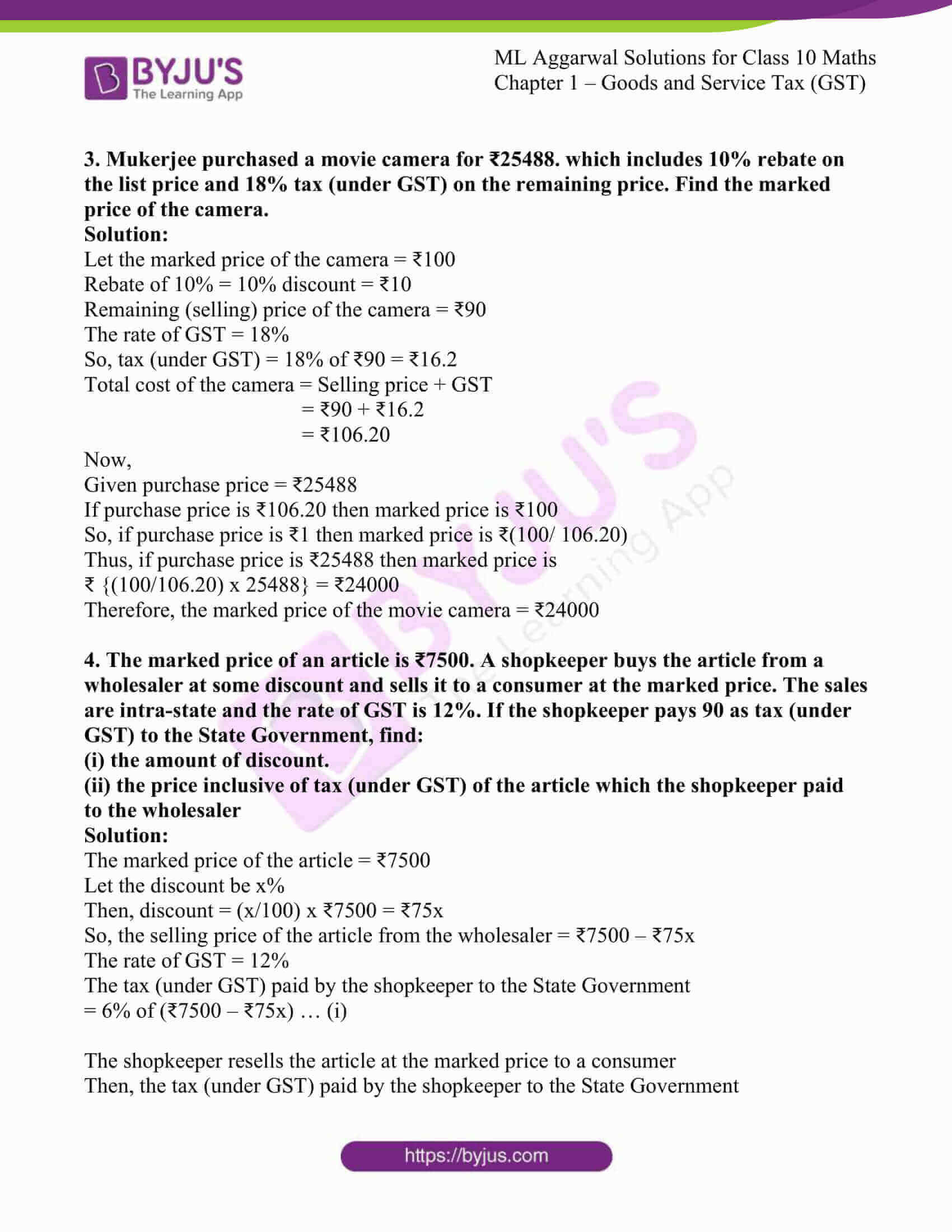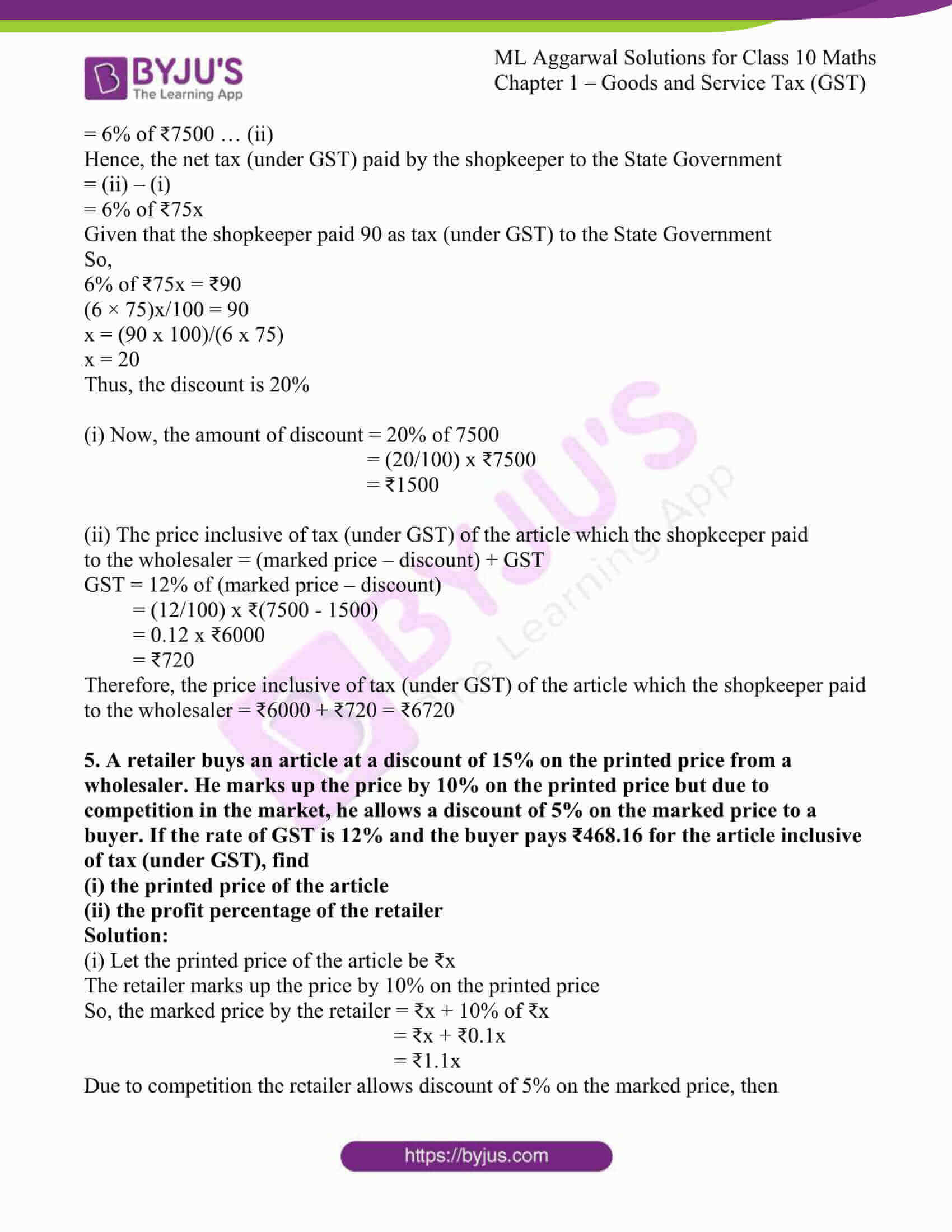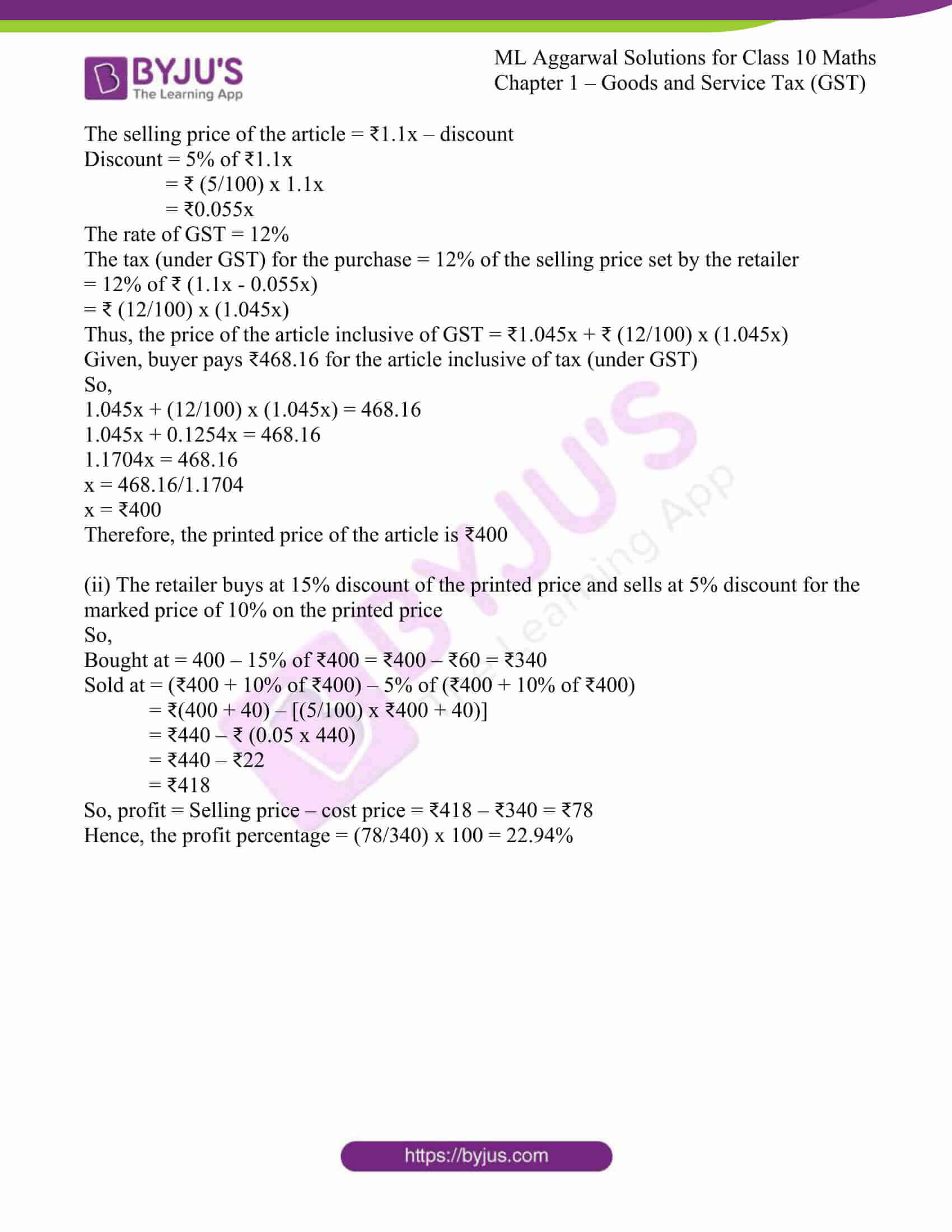### Access answers to ML Aggarwal Solutions for Class 10 Maths Chapter 1 – Goods and Service Tax (GST)

EXERCISE 1

1. An article is marked at 15000. A dealer sells it to a consumer at 10% profit. If the rate of GST is 12%, find:

(i) the selling price (excluding tax) of the article.

(ii) the amount of tax (under GST) paid by the consumer.

(iii) the total amount paid by the consumer.

Solution:

(i) Marked price of the article = ₹15000

When sold at 10% profit

Profit = (10/100) x ₹15000 = ₹1500

Thus, the selling price (excluding tax) = ₹15000 + ₹1500 = ₹16500

(ii) The rate of GST is 12%

Thus, the amount of tax (GST) paid by the consumer would be

= (12/100) x ₹16500

= ₹1980

(iii) Therefore, the total amount paid by the consumer = Selling price + GST

= ₹16500 + ₹1980

= ₹18480

2. A shopkeeper buy goods worth 4000 and sells these at a profit of 20% to a consumer in the same state. If GST is charged at 5%, find:

(i) the selling price (excluding tax) of the goods.

(ii) CGST paid by the consumer.

(iii) SGST paid by the consumer.

(iv) the total amount paid by the consumer.

Solution:

(i) Actual price of the goods = ₹4000

When sold at a profit of 20%

Profit = (20/100) x ₹4000 = ₹800

Thus, the selling price (excluding tax) of the goods will be

= Actual price + profit

= ₹4000 + ₹800

= ₹4800

The GST charged is 5%

(ii) CGST paid by the consumer = 2.5% of the selling price

= (2.5/100) x ₹4800

= ₹120

(iii) SGST paid by the consumer = 2.5% of the selling price

= (2.5/100) x ₹4800

= ₹120

(iv) Thus, the total amount paid by the consumer = selling price + CGST + SGST

= ₹4800 + ₹120 + ₹120

= ₹5040

3. The marked price of an article is 12500. A dealer in Kolkata sells the article to a consumer in the same city at a profit of 8%. If the rate of GST is 18%, find

(i) the selling price (excluding tax) of the goods.

(ii) IGST, CGST and SGST paid by the dealer to the Central and State Governments.

(iii) the amount which the consumer pays for the article

Solution:

(i)The marked price of the article = ₹12500

When sold at a profit of 8%

Profit = (8/100) x ₹12500 = ₹1000

Thus, the selling price (excluding tax) of the article = Marked price + profit

= ₹12500 + ₹1000

= ₹13500

(ii) The rate of GST = 18%

IGST is not applicable in this case since the dealing is intra state

CGST paid by the dealer to the Central Government = 9% of the selling price

= (9/100) x ₹13500

= ₹1215

And,

SGST paid by the dealer to the State Government = 9% of the selling price

= (9/100) x ₹13500

= ₹1215

(iii) Hence, the total amount which the consumer pays for the article will be

= Selling price + CGST + SGST

= ₹13500 + ₹1215 +
₹1215

= ₹15930

4. A shopkeeper buys an article from a wholesaler for ₹ 20000 and sells it to consumer at 10% profit. If the rate of GST is 12%, find the tax liability of the shopkeeper.

Solution:

Selling price of the wholesaler = ₹20000

Now, the cost price for the shopkeeper = ₹20000

When sold at a profit of 10%

Profit = (10/100) x ₹20000 = ₹2000

So, the selling of shopkeeper = Cost price + profit

= ₹20000 + ₹2000

= ₹22000

The rate of GST = 12 %

The tax liability of shopkeeper = Profit x GST %

= ₹2000 x (12/100)

=
₹240

5. A dealer buys an article for ₹ 6000 from a wholesaler. The dealer sells the article to a consumer at 15% profit. If the sales are intra-state and the rate of GST is 18%, find

(i) input CGST and input SGST paid by the dealer.

(ii) output CGST and output SGST collected by the dealer.

(iii) the net CGST and SGST paid by the dealer.

(iv) the total amount paid by the consumer.

Solution:

Selling price of the wholesaler = ₹6000

Cost price of the dealer = ₹6000

When sold at a profit of 15% to the consumer

Profit = (15/100) x ₹6000 = ₹900

So, the selling price of the dealer = Cost price + profit

= ₹6000 + ₹900

= ₹6900

Rate of GST = 18%

(i) Input CGST paid by the dealer = 9% of selling price of wholesaler

= (9/100) x ₹6000

= ₹540

And, input SGST paid by the dealer = 9% of selling price of wholesaler

= (9/100) x ₹6000

= ₹540

(ii) Output CGST collected by the dealer = 9% of selling price of the dealer

= (9/100) x ₹6900

= ₹621

And, output SGST collected by the dealer = 9% of selling price of the dealer

= (9/100) x ₹6900

= ₹621

(iii) Net CGST paid by the dealer = (Output CGST – Input CGST) paid by the dealer

= ₹621 – ₹540

= ₹81

And, Net SGST paid by the dealer = (Output SGST – Input SGST) paid by the dealer

= ₹621 – ₹540

= ₹81

(iv) The total amount paid by the consumer = Selling price of the dealer + CGST + SGST

= ₹6900 + ₹621 + ₹621

= ₹8142

6. A manufacture buys raw material worth ₹ 7500 paying GST at the rate of 5%. He sells the finished product to a dealer at 40% profit. If the purchased and the sale both are intra-state and the rate of GST for the finished product is 12%, find:

(i) the input tax (under GST) paid by the manufacturer.

(ii) the output tax (under GST) collected by the manufacturer.

(iii) the tax (under GST) paid by the manufacturer to the Central and State Governments.

(iv) the amount paid by the dealer for the finished product.

Solution:

(i) Cost of the raw material = ₹7500

The rate of GST = 5%

The input tax (under GST) paid by the manufacturer = 5% of ₹7500

= (5/100) x ₹7500

= ₹375

Thus, the CGST and SGST both are ₹187.50 each

(ii) The manufacture sells the finished product to a dealer at a profit of 40%

Profit = (40/100) x ₹7500

= ₹3000

Selling price = Cost price + profit

= ₹7500 + ₹3000

= ₹10500

The output tax (under GST) collected by the manufacture = 12% of the selling price

= (12/100) x ₹10500

= ₹1260

Thus, both CGST and SGST = ₹630

(iii) The net tax (under GST) paid by the manufacturer to the Central Government (CGST) = (Input – output) CGST

= ₹630 – ₹187.50

= ₹442.5

And, the net tax (under GST) paid by the manufacturer to the State Governments (SGST) = (Input – output) CGST

= ₹630 – ₹187.50

= ₹442.5

(iv) The amount paid by the dealer for the finished product = Selling price + GST

= ₹10500 + GST

= ₹10500 + (12/100) x ₹10500

= ₹10500 + ₹1260

= ₹11760

7. A manufacture sells a T.V to a dealer for Rs.18000 and the dealer sells it to a consumer at a profit of Rs 1500. If the sales are intra state and the rate of G.S.T is 12 %, Find:

(i) The amount of GST paid by the dealer to the State Government.

(ii) The amount of GST received by the Central Government.

(iii) The amount of GST received by the State Government.

(iv) The amount that the consumer pays for the TV.

Solution:

It is a case of intra-state transaction of goods and services.

SGST = CGST = ½ GST

Given:

Manufacturer sells T.V to a dealer = ₹ 18000

Amount of GST collected by manufacturer from dealer,

CGST – SGST = 6% of 18000

= (6/100) × 18000

= ₹ 1080

So, Manufacturer will pay ₹ 1080 as CGST and ₹ 1080 as SGST

CP of a TV for dealer = ₹ 18000

Profit = ₹ 1500

SP of a TV for dealer to customer – CP + Profit = ₹ 18000 + ₹ 1500

= ₹ 19500

Amount of GST collected by dealer from customer,

CGST = SGST = 6% of ₹ 19500

= (6/100) × 19500

= ₹ 1170

(i) Amount of GST paid by the dealer to the State Government.

₹ 1170 – ₹ 1080 = ₹ 90

(ii) Amount of GST received by the Central Government.

CGST paid by manufacturer + CGST paid by dealer = ₹ 1080 + ₹ 90

= ₹ 1170

(iii) Amount of GST received by the State Government.

SGST paid by manufacturer + SGST paid by dealer = ₹ 1080 + ₹ 90

= ₹ 1170

(iv) Amount that the consumer pays for the TV.

CP of TV + CGST paid by customer + SGST paid by customer

= ₹19500 + ₹1170 + ₹ 1170 = ₹ 21840

8. A shopkeeper buys a camera at a discount of 20% from a wholesaler. The printed price of the camera being Rs 1600. The shopkeeper tells it to a consumer at the printed price.

If the sales are intra-state and the rate of GST Is 12%, find:

(i) GST paid by the shopkeeper to the Central Government

(ii) GST received by the Central Government.

(iii) GST received by the State Government.

(iv) The amount at which the consumer bought the camera.

Solution:

It is a case of intra-state transaction of goods and services.

SGST = CGST = ½ GST

Given:

Printed price of a camera = ₹ 1600

Rate of discount = 20%

CP of camera for shopkeeper = printed price – Discount

= ₹1600 – 20% of ₹1600

= ₹1600 – (20/100) × 1600

= ₹1600 – ₹320

= ₹1280

It is given that, rate of GST = 12%

Amount of GST paid by the shopkeeper to the wholesaler,

CGST = SGST = 6% of ₹1280

= (6/100) × 1280

= ₹76.80

(i) GST paid by the shopkeeper to the Central Government

CGST = SGST = 6% of ₹1600

= (6/100) × 1600

= ₹96

GST paid by the shopkeeper to the Central Government = ₹96 – ₹76.80 = ₹19.20

(ii) GST received by the Central Government.

CGST paid by wholesaler + CGST paid by shopkeeper = ₹76.80 + ₹19.20 = ₹96

(iii) GST received by the State Government.

SGST paid by wholesaler + SGST paid by shopkeeper = ₹76.80 + ₹19.20 = ₹96

(iv) The amount at which the consumer bought the camera.

Amount paid by consumer for camera = CP of camera + CGST paid by consumer + SGST paid by consumer = ₹1600 + ₹96 + ₹96 = ₹1792

9. A dealer buys an article at a discount of 30% from the wholesaler, the marked price being Rs 6000. The dealer sells it to a consumer at a discount of 10% on the marked price. If the sales are intra-state and the rate of GST is 5%. Find:

(i) The amount paid by the consumer for the article.

(ii) The tax (under GST) paid by the dealer to the State Government.

(iii) The amount of tax (under GST) received by the Central Government.

Solution:

It is a case of intra-state transaction of goods and services.

SGST = CGST = ½ GST

Given:

Marked price of an article = ₹6000

Rate of GST = 5%

Rate of discount given by the wholesaler = 30%

CP of an article for dealer = Marked price – Discount

= ₹6000 – 30% of ₹6000

= ₹6000 – (30/100) × 6000

= ₹6000 – 1800

= ₹4200

Amount of GST paid by dealer to wholesaler,

CGST = SGST = 2.5% of ₹4200

= (2.5/100) × 4200

= ₹105

(i) The amount paid by the consumer for the article.

SP of an article for consumer = Marked price – Discount

= ₹6000 – 10% of ₹6000

= ₹6000 – (10/100) × 6000

= ₹6000 – 600

= ₹5400

Amount of GST paid by consumer to dealer,

CGST = SGST = 2.5% of ₹5400

= (2.5/100) × 5400

= ₹135

Amount paid by consumer for article = CP of article for consumer + CGST paid by consumer + SGST paid by consumer = ₹5400 + ₹135 + ₹135 = ₹5670

(ii) The tax (under GST) paid by the dealer to the State Government.

₹135 – ₹105 = ₹30

(iii) The amount of tax (under GST) received by the Central Government.

CGST paid by wholesaler + CGST by dealer = ₹105 + ₹30 = ₹135

10. The printed price of an article is Rs 50000. The wholesaler allows a discount of 10% to a shopkeeper. The shopkeeper sells the article to a consumer at 4% above the marked price. If the sales are intra-state and the rate of GST is 18%, find:

(i) The amount inclusive of tax (under GST) which the shopkeeper pays for the articles.

(ii) The amount paid by the consumer for the article.

(iii) The amount of tax (under GST) paid by the shopkeeper to the Central Government.

(iv) The amount of tax (under GST) received by the State Government.

Solution:

It is a case of intra-state transaction of goods and services.

SGST = CGST = ½ GST

Given:

Marked price of an article = ₹50000

Rate of GST = 18%

(i) The amount inclusive of tax (under GST) which the shopkeeper pays for the articles.

Rate of discount given by the wholesaler = 10%

CP of an article for shopkeeper = Marked price – Discount

= ₹50000 – 10% of ₹50000

= ₹50000 – (10/100) × 50000

= ₹50000 – 5000

= ₹45000

Amount of GST paid by dealer to wholesaler,

CGST = SGST = 9% of ₹45000

= (9/100) × 45000

= ₹4050

Amount paid by shopkeeper for an article = CP of an article for shopkeeper + CGST paid by consumer + SGST paid by consumer = ₹45000 + ₹4050 + ₹4050 = ₹53100

(ii) The amount paid by the consumer for the article.

SP of an article for consumer = Marked price – Discount

= ₹50000 – 4% of ₹50000

= ₹50000 – (4/100) × 50000

= ₹50000 – 2000

= ₹48000

Amount of GST paid by consumer to dealer,

CGST = SGST = 9% of ₹48000

= (9/100) × 48000

= ₹4320

Amount paid by consumer for article = CP of article for consumer + CGST paid by consumer + SGST paid by consumer = ₹48000 + ₹4320 + ₹4320 = ₹56640

(iii) The amount of tax (under GST) paid by the shopkeeper to the Central Government.

₹4320 – ₹4050 = ₹270

(iv) The amount of tax (under GST) received by the State Government.

SGST paid by wholesaler + SGST paid by shopkeeper = ₹4050 + ₹270 = ₹4320

11. A retailer buys a TV from a wholesaler for Rs 40000. He marks the price of the T.V. 15% above his cost price and sells it to a consumer at 5% discount on the marked price. If the sales are intra-state and the rate of GST is 12%, find:

(i) The marked price of the TV.

(ii) The amount which the consumer pays for the TV.

(iii) The amount of tax (under GST) paid by the retailer to the Central Government.

(iv) The amount of tax (under GST) received by the State Government.

Solution:

It is a case of intra-state transaction of goods and services.

SGST = CGST = ½ GST

Given:

(i) The marked price of the TV.

It is given that, CP of TV for retailer = ₹40000

Marked price of TV = ₹40000 + 15% of 40000

= ₹40000 + (15/100) × 40000

= ₹40000 + 6000

= ₹46000

(ii) The amount which the consumer pays for the TV.

It is given that, Discount given by retailer = 5% of ₹46000

= (5/100) × 46000

= ₹2300

Amount paid by consumer without GST for TV = ₹46000 – ₹2300

= ₹43700

Rate of GST = 12%

Amount of GST paid by consumer = 12% of ₹43700

= (12/100) × 43700

= ₹5244

Amount which consumer pays for TV = ₹43700 + ₹5244 = ₹48944

(iii) The amount of tax (under GST) paid by the retailer to the Central Government.

CGST paid by shopkeeper = 6% of ₹40000

= (6/100) × 40000

= ₹2400

SGST paid by shopkeeper = 6% of ₹40000 = ₹2400

Shopkeeper sells the article to consumer = ₹43700

GST collected by shopkeeper = 12% of ₹43700

= (12/100) × 43700

= ₹5244

CGST of shopkeeper = SGST = 6% of ₹43700

= (6/100) × 43700

= ₹2622

The amount of tax (under GST) paid by the retailer to the Central Government =

₹2622 – ₹2400 = ₹222

(iv) The amount of tax (under GST) received by the State Government.

SGST paid by wholesaler + SGST paid by shopkeeper = ₹2400 + ₹222 = ₹2622

12. A shopkeeper buys an article from a manufacturer for Rs 12000 and marks up it price by 25%. The shopkeeper gives a discount of 10% on the marked up price and he gives a further off-season discount of 5% or, the balance to a customer of TV. If the sales are intra-state and the rate of CST is 12%, find:

(i) The price inclusive of tax (under GST) which the consumer pays for the TV.

(ii) The amount of tax (under GST) paid by the shopkeeper to the Stale Government.

(iii) The amount of tax (under CST) received by the Central Government.

Solution:

It is a case of intra-state transaction of goods and services.

SGST = CGST = ½ GST

Given:

(i) The price inclusive of tax (under GST) which the consumer pays for the TV.

CP of an article for shopkeeper = ₹12000

Marked price of article = ₹12000 + 25% of ₹12000

= ₹12000 + (25/100) × 12000

= ₹15000

Amount of discount given by shopkeeper = 10% of ₹15000

= (10/100) × 15000

= ₹1500

Again, shopkeeper gives off season discount of 5% on the balance = 5% of (15000 – 1500) = (5/100) × 13500

= ₹675

CP of TV for consumer = ₹13500 – ₹675 = ₹12825

Amount of GST paid by consumer = 12% of ₹12825

= (12/100) × 12825

= ₹1539

The price inclusive of tax (under GST) which the consumer pays for the TV = ₹12825 + ₹1539 = ₹14364

(ii) The amount of tax (under GST) paid by the shopkeeper to the Stale Government.

CGST = SGST = 6% of ₹12000

= (6/100) × 12000

= ₹720

GST paid by consumer to shopkeeper,

CGST = SGST = 6% of ₹12825

= (6/100) × 12825

= ₹769.50

The amount of tax (under GST) paid by the shopkeeper to the Stale Government =

₹769.50 – ₹720 = ₹49.50

(iii) The amount of tax (under CST) received by the Central Government.

CGST paid by manufacturer = ₹720

CGST paid by shopkeeper = ₹769.50 – ₹720 = ₹49.50

The amount of tax (under CST) received by the Central Government =

₹720 + ₹49.50 = ₹769.50

13. The printed price of an article is Rs 40000. A wholesaler in Uttar Pradesh buys the article horn a manufacturer in Gujarat at a discount of 10% on the printed price. The wholesaler sells the article to a retailer in Himachal at 5% above the printed price. If the rate of GST on the article is 18%, find:

(i) The amount inclusive of tax (under GST) paid by the wholesaler for the article.

(ii) The amount inclusive of tax (under GST) paid by the retailer for the article.

(iii) The amount of tax (under GST) paid by the wholesaler to the Central Government.

(iv) The amount of tax (under GST) received by the Central Government.

Solution:

Here, both given sales from manufacturer to wholesaler and wholesaler to retailer are inter-state.

So, CGST = SGST = 0

GST = IGST

Given:

Printed price of an article = ₹40000

Discount given by manufacturer = 10% of ₹40000

= (10/100) × 40000

= ₹4000

CP of article for wholesaler = ₹40000 – ₹4000 = ₹36000

CP of article without tax for retailer = ₹40000 + 5% of ₹40000

= ₹40000 + (5/100) × 40000

= ₹42000

(i) The amount inclusive of tax (under GST) paid by the wholesaler for the article.

Amount of GST paid by wholesaler to manufacturer = 18% of ₹36000

= (18/100) × 36000

= ₹6480

The amount inclusive of tax (under GST) paid by the wholesaler for the article =

₹36000 + ₹6480 = ₹42480

(ii) The amount inclusive of tax (under GST) paid by the retailer for the article.

Amount of GST paid by retailer to wholesaler = 18% of ₹42000

= (18/100) × 42000

= ₹7560

The amount inclusive of tax (under GST) paid by the retailer for the article =

₹42000 + ₹7560 = ₹49560

(iii) The amount of tax (under GST) paid by the wholesaler to the Central Government.

Amount of GST paid by wholesaler to manufacturer = 18% of ₹36000

= (18/100) × 36000

= ₹6480

Amount of GST paid by retailer to wholesaler = 18% of ₹42000

= (18/100) × 42000

= ₹7560

The amount of tax (under GST) paid by the wholesaler to the Central Government =

₹7560 – ₹6480 = ₹1080

(iv) The amount of tax (under GST) received by the Central Government.

IGST paid by wholesaler to the Central Government = ₹1080

IGST paid by manufacturer = ₹6480

The amount of tax (under GST) received by the Central Government = ₹1080 + ₹6480

= ₹7560

14. A dealer in Delhi buys an article for ₹16000 from a wholesaler in Delhi. He sells the article to a consumer in Rajasthan at a profit of 25%. If rate of GST is 5%, find:

(i) the tax (under GST) paid by the wholesaler to Government.

(ii) the tax (under GST) paid by the dealer to the Government.

(iii) the amount which the consumer pay for the article.

Solution:

Cost price of the article for the dealer = 16000

As the sales are interstate the GST will go the central government

GST = (5/100) × 16000

= ₹800

The article is sold at a profit of 25% to a consumer

Profit = (25/100) × 16000

= ₹4000

Thus, the selling price of the article = 16000 + 4000 = ₹20000

As the sales are interstate, then

GST = 5/100 × ₹20000

= ₹1000

(i) The tax paid by the wholesaler to the governments

= ₹800/2

= ₹400

Thus, CGST = ₹400 and SGST = ₹400

(ii) The tax paid by the dealer to the governments

= IGST = ₹1000 – ₹800 = ₹200

(iii) Total amount paid by the consumer for the article

= 20000 + 1000

= ₹21000

15. A shopkeeper in Delhi buys an article at the printed price of Rs 24000 from a wholesaler in Mumbai. The shopkeeper sells the article to a consumer in Delhi at a profit of 15% on the basic cost price. if the rate of GST is 12%, find:

(i) The price inclusive of tax (under GST) at which the wholesaler bought the article.

(ii) The amount which the consumer pays for the article.

(iii) The amount of tax (under GST) received by the State Government of Delhi.

(iv) The amount of tax (under GST) received by the Central Government.

Solution:

Given:

(i) The price inclusive of tax (under GST) at which the wholesaler bought the article.

CP of an article for shopkeeper = ₹24000

Rate of GST = 12%

IGST collected by wholesaler from shopkeeper = 12% of ₹24000

= (12/100) × 24000

= ₹2880

The price inclusive of tax (under GST) at which the wholesaler bought the article =

CP of article for shopkeeper + IGST paid by shopkeeper to wholesaler = ₹24000 + ₹2880

= ₹26880

(ii) The amount which the consumer pays for the article.

CP of an article for shopkeeper = ₹24000

Profit on CP of article = 15% of CP

SP of an article by the shopkeeper to consumer = CP + Profit

= ₹24000 + 15% of ₹24000

= ₹24000 + (15/100) × 24000

= ₹24000 + 3600

= ₹27600

The amount which the consumer pays for the article = CP of article for consumer + CGST paid by the consumer + SGST paid by consumer =

₹27600 + 6% of ₹27600 + 6% of ₹27600 =

₹27600 + (6/100) × ₹27600 + (6/100) × ₹27600 = ₹27600 + ₹1656 + ₹1656

= ₹30912

(iii) The amount of tax (under GST) received by the State Government of Delhi.

Amount of IGST for shopkeeper = ₹2880

SP of an article to consumer = CP of article for shopkeeper + profit on basic CP

= ₹24000 + 15% of ₹24000

= ₹24000 + (15/100) × ₹24000

= ₹24000 + ₹3600

= ₹27600

As the shopkeeper sells an article to consumer in Delhi; so this sales is Intra-state sales.

Amount of GST collected by shopkeeper from consumer,

CGST = SGST = 6% of ₹27600

= (6/100) × ₹27600

= ₹1656

Amount of tax paid by shopkeeper to state govt. = ₹2880 – ₹1656 = ₹1224

The amount of tax (under GST) received by the State Government of Delhi =

₹1656 – ₹1224 = ₹432

(iv) The amount of tax (under GST) received by the Central Government.

The amount of tax (under GST) received by the Central Government = IGST received from wholesaler + CGST received from shopkeeper = ₹ 2880 + NIL = ₹ 2880

16. A dealer in Maharashtra buys an article from a wholesaler in Maharashtra at a discount of 25%, the printed price of the article being ₹ 20000. He sells the article to a consumer in Telangana at a discount of 10% on the printed price. If the rate of GST is 12%, find

(i) the tax (under GST) paid by the wholesaler to Governments.

(ii) the tax (under GST) paid by the dealer to the Governments.

(iii) the amount which the consumer pays for the article.

Solution:

The printed price of the article = ₹2000

Discount rate = 25%

Discount = (25/100) x ₹20000 = ₹5000

So, the selling price of the article by the wholesaler = Printed price – discount

= ₹20000 – ₹5000

= ₹15000

(i) The tax (under GST) paid by the wholesaler to the Governments = 12% of the selling price

= (12/100) x ₹1500

= ₹1800

So, the CGST = ₹900 and SGST = ₹900

And, the total amount paid by the dealer = selling price + GST

= ₹15000 + ₹1800

= ₹16800

(ii) The dealer resells the article at a discount of 10% from the marked price

So, the discount = (10/100) x ₹20000

= ₹2000

Hence, the selling of the article from the dealer = marked price – discount

= ₹20000 – ₹2000

= ₹18000

Now, the tax (under GST) paid by the dealer to the Governments = 12% of the selling price

IGST = (12/100) x ₹18000

= ₹2160

Thus, the net tax (under GST) paid by the dealer to the governments = ₹2160 – ₹1800

= ₹360

(iii) The amount which the consumer pays for the article = Selling price of the dealer + IGST

= ₹18000 + 12% of ₹18000

= ₹18000 + ₹2160

= ₹20160

17. Kiran purchases an article for Rs 5310 which includes 10% rebate on the marked price and 18% tax (under GST) on the remaining price. Find the marked price of the article.

Solution:

Given:

Rate of GST = 18%

CP of an article = x – 10% of x

= x – (10/100)x

= 90x/100

= 9x/10

Amount of GST on CP of article = 18% of 9x/10

= (18/100) × 9x/10

Total CP of article = 9x/10 + [(18/100) × 9x/10] – 9x/10(1 + 18/100) – (118/100) × 9x/10

It is given that, CP of an article including tax = ₹5310

So,

(118/100) × 9x/10 = 5310

x = 5310 × (100/118) × (10/9)

= 5000

The required marked price of an article is ₹5000

18. A Shopkeeper buys an article whose list price is Rs 8000 at some rate of discount from the wholesaler. He sells the article to a consumer at the list price. The sales are intra-state and the rate of GST is 18%. If the shopkeeper pays a tax (under GST) of 72 to the State Government, find the rate of discount at which he bought the article from the wholesaler.

Solution:

Given:

List of price of an article = ₹8000

Let the rate of discount given by wholesaler = x%

So,

Discount = x% of ₹8000

= (x/100) × ₹8000

= ₹80x

CP of an article for shopkeeper = ₹8000 – ₹80x

It is given that, CP of article for consumer = ₹8000

Since the sales are intra-state, rate of GST = 18%

CGST = SGST = 9%

Amount of GST paid by shopkeeper to wholesaler,

SGST = CGST = 9% of [₹8000 – ₹80x]

= (9/100) × [₹8000 – ₹80x]

Amount of GST paid by consumer to shopkeeper,

CGST = SGST = 9% of ₹8000

= (9/100) × ₹8000

= ₹720

So, the tax paid by shopkeeper to state government = ₹720 – (9/100) × [₹8000 – ₹80x]

Also, tax paid by shopkeeper to state government = ₹72

₹72 – 720 – ((9×80)/100) (100 – x)

720 – 72 = (720/100) (100 – x)

648 = (72/10) (100 – x)

100 – x = (648×10)/72

100 – x = 90

x = 100 – 90

= 10

Hence, the required rate of discount = 10%

Chapter Test

1. A shopkeeper bought a washing machine at a discount of 20% from a wholesaler, the printed price of the washing machine being ₹ 18000. The shopkeeper sells it to a consumer at a discount of 10% on the printed price. If the sales are intra-state and the rate of GST is 12%, find:

(i) the price inclusive of tax (under GST) at which the shopkeeper bought the machine

(ii) the price which the consumer pays for the machine

(iii) the tax (under GST) paid by the wholesaler to the State Government

(iv) the tax (under GST) paid by the shopkeeper to the State Government

(v) the tax (under GST) received by the Central Government

Solution:

Printed Price of the washing machine = ₹18000

Discount rate = 20 %

Discount = (20/100) x ₹18000

= ₹3600

So, the selling price of the washing machine = ₹18000 – ₹3600 = ₹14400

The rate of GST = 12%

The taxes (under GST) for the purchase are

SGST = ₹14400 x (12/2)/100 = ₹864

CGST = ₹14400 x (12/2)/100 = ₹864

(i) Hence, the shopkeeper bought the machine at the price = ₹14400 + ₹864 + ₹864 = ₹16128

(iii) The tax (under GST) paid by wholesaler to State Government = ₹864

Now,

The machine is sold to a consumer at 10% discount of the printed rice

Discount = (10/100) x ₹18000

= ₹1800

So, the selling price for the shopkeeper = ₹18000 – ₹1800 = ₹16200

The taxes (under GST) for the purchase are

SGST = ₹16200 x (12/2)/100 = ₹972

CGST = ₹16200 x (12/2)/100 = ₹972

(ii) Thus, the consumer paid a price = ₹16200 + ₹972 + ₹972 = ₹18144

Now,

(iv) The tax (under GST) paid by shopkeeper to State Government = ₹972 – ₹864 = ₹108

And,

(v) The tax (under GST) received by the central Govt = ₹972

2. A manufacturer listed the price of his goods at 1600 per article. He allowed a discount of 25% to a wholesaler who in turn allowed a discount of 20% on the listed price to a retailer. The retailer sells one article to a consumer at a discount of 5% on the listed price. If the sales are intrastate and the rate of GST is 5%, find:
(i) the price per article inclusive of tax (under GST) which the wholesaler pays.
(ii) the price per article inclusive of tax (under GST) which the retailer pays.
(iii) the amount which the consumer pays for the article
(iv) the tax (under GST) paid by the wholesaler to the State Government for the article.
(v) the tax (under GST) paid by the retailer to the Central Government for the article.
(vi) the tax (under GST) received by the State Government.

Solution:

(i) The listed price per article = ₹1600

Discount rate from the manufacture = 25%

Discount = (25/100) x ₹1600 = ₹400

So, the selling price per article to the wholesaler = listed price – discount

= ₹1600 – ₹400

= ₹1200

The rate of GST = 5%

GST = 5% of ₹1200

= (5/100) x ₹1200

= ₹60

Thus, the price per article inclusive of tax (under GST) which the wholesaler pays = selling price of the manufacture + GST

= ₹1200 + ₹60

= ₹1260

(ii) The wholesaler resells at a discount of 20% on the listed price per article to the retailer

Discount = (20/100) x ₹1600

= ₹320

So, the selling price of the wholesaler = listed price – discount

= ₹1600 – ₹320

= ₹1280

The rate of GST = 5%

GST = 5% of ₹1280

= (5/100) x ₹1280

= ₹64

Thus, the price per article inclusive of tax (under GST) which the retailer pays = selling price of the wholesaler + GST

= ₹1280 + ₹64

= ₹1344

(iii) Further, the retailer resells at a discount of 5% on the listed per article to the consumer

Discount = (5/100) x ₹1600

= ₹80

So, the selling price of the wholesaler = listed price – discount

= ₹1600 – ₹80

= ₹1520

The rate of GST = 5%

GST = 5% of ₹1520

= (5/100) x ₹1520

= ₹76

Thus, the price per article inclusive of tax (under GST) which the consumer pays = selling price of the retailer + GST

= ₹1520 + ₹76

= ₹1596

(iv) The tax (under GST) paid by the wholesaler to the State Government for the article = ₹(64 – 62)/2

= ₹4/2

= 2

(v) The tax (under GST) paid by the retailer to the Central Government for the article = ₹ (76 – 84)/2

= ₹12/2

= ₹6

(vi) The tax (under GST) received by the State Government = ₹76/2 = ₹38

3. Mukerjee purchased a movie camera for ₹25488. which includes 10% rebate on the list price and 18% tax (under GST) on the remaining price. Find the marked price of the camera.

Solution:

Let the marked price of the camera = ₹100

Rebate of 10% = 10% discount = ₹10

Remaining (selling) price of the camera = ₹90

The rate of GST = 18%

So, tax (under GST) = 18% of ₹90 = ₹16.2

Total cost of the camera = Selling price + GST

= ₹90 + ₹16.2

= ₹106.20

Now,

Given purchase price = ₹25488

If purchase price is ₹106.20 then marked price is ₹100

So, if purchase price is ₹1 then marked price is ₹(100/ 106.20)

Thus, if purchase price is ₹25488 then marked price is

₹ {(100/106.20) x 25488} = ₹24000

Therefore, the marked price of the movie camera = ₹24000

4. The marked price of an article is ₹7500. A shopkeeper buys the article from a wholesaler at some discount and sells it to a consumer at the marked price. The sales are intra-state and the rate of GST is 12%. If the shopkeeper pays 90 as tax (under GST) to the State Government, find:

(i) the amount of discount.
(ii) the price inclusive of tax (under GST) of the article which the shopkeeper paid
to the wholesaler

Solution:

The marked price of the article = ₹7500

Let the discount be x%

Then, discount = (x/100) x ₹7500 = ₹75x

So, the selling price of the article from the wholesaler = ₹7500 – ₹75x

The rate of GST = 12%

The tax (under GST) paid by the shopkeeper to the State Government

= 6% of (₹7500 – ₹75x) … (i)

The shopkeeper resells the article at the marked price to a consumer

Then, the tax (under GST) paid by the shopkeeper to the State Government

= 6% of ₹7500 … (ii)

Hence, the net tax (under GST) paid by the shopkeeper to the State Government

= (ii) – (i)

= 6% of ₹75x

Given that the shopkeeper paid 90 as tax (under GST) to the State Government

So,

6% of ₹75x = ₹90

(6 × 75)x/100 = 90

x = (90 x 100)/(6 x 75)

x = 20

Thus, the discount is 20%

(i) Now, the amount of discount = 20% of 7500

= (20/100) x ₹7500

= ₹1500

(ii) The price inclusive of tax (under GST) of the article which the shopkeeper paid

to the wholesaler = (marked price – discount) + GST

GST = 12% of (marked price – discount)

= (12/100) x ₹(7500 – 1500)

= 0.12 x ₹6000

= ₹720

Therefore, the price inclusive of tax (under GST) of the article which the shopkeeper paid to the wholesaler = ₹6000 + ₹720 = ₹6720

5. A retailer buys an article at a discount of 15% on the printed price from a wholesaler. He marks up the price by 10% on the printed price but due to competition in the market, he allows a discount of 5% on the marked price to a buyer. If the rate of GST is 12% and the buyer pays ₹468.16 for the article inclusive of tax (under GST), find

(i) the printed price of the article

(ii) the profit percentage of the retailer

Solution:

(i) Let the printed price of the article be ₹x

The retailer marks up the price by 10% on the printed price

So, the marked price by the retailer = ₹x + 10% of ₹x

= ₹x + ₹0.1x

= ₹1.1x

Due to competition the retailer allows discount of 5% on the marked price, then

The selling price of the article = ₹1.1x – discount

Discount = 5% of ₹1.1x

= ₹ (5/100) x 1.1x

= ₹0.055x

The rate of GST = 12%

The tax (under GST) for the purchase = 12% of the selling price set by the retailer

= 12% of ₹ (1.1x – 0.055x)

= ₹ (12/100) x (1.045x)

Thus, the price of the article inclusive of GST = ₹1.045x + ₹ (12/100) x (1.045x)

Given, buyer pays ₹468.16 for the article inclusive of tax (under GST)

So,

1.045x + (12/100) x (1.045x) = 468.16

1.045x + 0.1254x = 468.16

1.1704x = 468.16

x = 468.16/1.1704

x = ₹400

Therefore, the printed price of the article is ₹400

(ii) The retailer buys at 15% discount of the printed price and sells at 5% discount for the marked price of 10% on the printed price

So,

Bought at = 400 – 15% of ₹400 = ₹400 – ₹60 = ₹340

Sold at = (₹400 + 10% of ₹400) – 5% of (₹400 + 10% of ₹400)

= ₹(400 + 40) – [(5/100) x ₹400 + 40)]

= ₹440 – ₹ (0.05 x 440)

= ₹440 – ₹22

= ₹418

So, profit = Selling price – cost price = ₹418 – ₹340 = ₹78

Hence, the profit percentage = (78/340) x 100 = 22.94%